# US4760378A - Method and apparatus for converting a run length limited code - Google Patents

Method and apparatus for converting a run length limited code Download PDF

## Info

Publication number
US4760378A
US4760378A US06/719,629 US71962985A US4760378A US 4760378 A US4760378 A US 4760378A US 71962985 A US71962985 A US 71962985A US 4760378 A US4760378 A US 4760378A
Authority
US
United States
Prior art keywords
code
word
code word
words
max
Prior art date
Legal status (The legal status is an assumption and is not a legal conclusion. Google has not performed a legal analysis and makes no representation as to the accuracy of the status listed.)
Application number
US06/719,629
Inventor
Akira Iketani
Chojuro Yamamitsu
Ichiro Ogura
Current Assignee (The listed assignees may be inaccurate. Google has not performed a legal analysis and makes no representation or warranty as to the accuracy of the list.)
Panasonic Corp
Original Assignee
Panasonic Corp
Priority date (The priority date is an assumption and is not a legal conclusion. Google has not performed a legal analysis and makes no representation as to the accuracy of the date listed.)
Filing date
Publication date
Priority to JP59-102138 priority Critical
Priority to JP10213884A priority patent/JPS60246155A/en
Priority to JP59186705A priority patent/JPS6165531A/en
Priority to JP59-186705 priority
Application filed by Panasonic Corp filed Critical Panasonic Corp
Assigned to MATSUSHITA ELECTRIC INDUSTRIAL CO., LTD., A CORP. OF JAPAN reassignment MATSUSHITA ELECTRIC INDUSTRIAL CO., LTD., A CORP. OF JAPAN ASSIGNMENT OF ASSIGNORS INTEREST. Assignors: IKETANI, AKIRA, OGURA, ICHIRO, SUESADA, KUNIO, YAMAMITSU, CHOJURO
Publication of US4760378A publication Critical patent/US4760378A/en
Application granted granted Critical
Anticipated expiration legal-status Critical
Application status is Expired - Lifetime legal-status Critical

## Images

•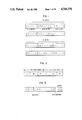•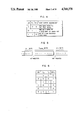•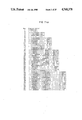•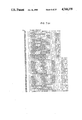•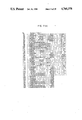•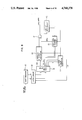•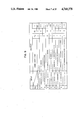•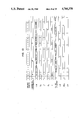•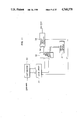•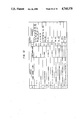••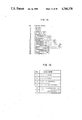••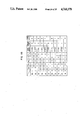••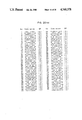••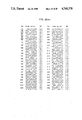•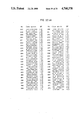•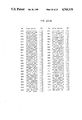•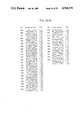•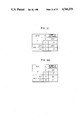•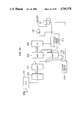•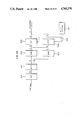•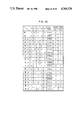•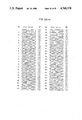•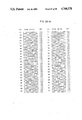•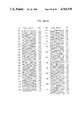•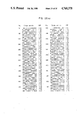•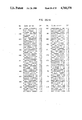•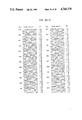•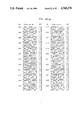•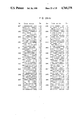•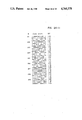•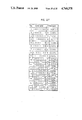## Classifications

• GPHYSICS
• G11INFORMATION STORAGE
• G11BINFORMATION STORAGE BASED ON RELATIVE MOVEMENT BETWEEN RECORD CARRIER AND TRANSDUCER
• G11B20/00Signal processing not specific to the method of recording or reproducing; Circuits therefor
• G11B20/10Digital recording or reproducing
• G11B20/14Digital recording or reproducing using self-clocking codes
• G11B20/1403Digital recording or reproducing using self-clocking codes characterised by the use of two levels
• G11B20/1423Code representation depending on subsequent bits, e.g. delay modulation, double density code, Miller code
• G11B20/1426Code representation depending on subsequent bits, e.g. delay modulation, double density code, Miller code conversion to or from block codes or representations thereof

## Abstract

A systematic method and apparatus for constructing a run length limited code in which the minimum number of continuous bits of the same binary value is constrained to d and the maximum number thereof is constrained to k.
In converting m-bit data words to n-bit code words (n>m) to construct the run length limited code, selection means for n-bit code words usable to meet the d, k-constraint and a concatenation rule of the code words selected by the selection means are introduced.
The selection means divides each of 2n n-bit bit sequences into a leading block L having l continuous bits of the same binary value, an end block R having γ continuous bits of the same binary value and an intermediate block B having b(=n-l-γ) bits between the blocks L and R.
Only those n-bit bit sequences in which the blocks B thereof completely meet the d, k-constraint and the blocks L and R thereof meet conditions uniquely defined for given d and k are used as the code words. Consequently, a systematic method for constructing the run length limited code is provided.

## Description

BACKGROUND OF THE INVENTION

The present invention relates to method and apparatus for converting a run length limited (RLL) code for converting m-bit data words to n-bit code words while constraining the minimum number of continuous bits having the same binary value to d and the maximum number of continuous bits having the same binary value to k in a bit sequence generated by concatenation of the code words.

The RLL code is usually used in recording digital data at a high record density on a magnetic tape or a magnetic disk.

The RLL code is defined as the code in which a minimum number of continuous bits having the same binary value is constrained to d and the maximum number of continuous bits having the same binary value is constrained to k. The RLL code having such a property is generated by converting m-bit data words (each having a bit length of T) to n-bit code words, where n is larger than m.

In such an RLL code, the interval Tw required to identify one bit (hereinafter referred to as a detection window) is m/nT and the minimum interval between transitions Tmin is d·Tw.

In a recording and reproducing system, intersymbol interference usually occurs because high frequency components are cut off. In order to minimize the intersymbol interference, it is desirable for Tmin to be long. In order to suppress an influence by a time-axis variation such as a peak shift and jitter due to the intersymbol interference, it is desirable for the detection window Tw to be long. In addition, in order to attain a self-clocking function, it is desirable for the maximum number of continuous bits k to be small.

In view of the above, various RLL codes have been developed such the 8/10 conversion code (ref. No. 1), the 8/9 conversion code (ref. No. 2), the 8/16 conversion code (ref. No. 3), the 2/3 conversion code (ref. No. 4), the 3PM code (ref. No. 5), the HDM-3 code (ref. No. 6) and the (2, 7) RLLC code (ref. No. 7). These references are identified below.

The 8/10 conversion code is an RLL code in which d=1, k=10, m=8, n=10, Tw =0.8T and Tmin =0.8T in accordance with the above definitions.

The 8/9 conversion code is an RLL code in which d=1, k=14, m=8, n=9, Tw =8/9T and Tmin =8/9T.

The 8/16 conversion code is an RLL code in which d=2, k=6, m=8, n=16, Tw =0.5T and Tmin =T.

Those three RLL codes are DC free codes which do not include a D.C. component and in which Tw has a larger weight than Tmin.

Since those RLL codes were developed primarily for a digital VTR in which low frequency components are cut off by a rotary transformer, they are DC free in nature and have a large Tw because of a requirement for an extremely high recording density. On the other hand, those RLL codes have a large k.

The DC free code is defined as a code in which the difference between the number of "1"s and the number of "0"s included between any two bits in a bit sequence generated by concatenation of code words is definite. Digital Sum Variation (DSV) is referred to as the variation in the running sum of a bit sequence after conversion and the difference between the number of "1"s and the number of "0"s in the code word is referred to as disparity (DP).

On the other hand, the 2/3 conversion code is a variable length RLL code (ref. No. 7) in which d=2, k=8, m=2, n=3, Tw =2/3T, and Tmin =4/3T in accordance with the above definitions.

The 3PM code is an RLL code in which d=3, k=12, m=3, n=6, Tw =0.5T and Tmin =1.5T.

The HDM-3 code is an RLL code in which d=6, k=25, m=4, n=12, Tw =T/3 and Tmin =2T.

The (2, 7) RLLC code is a variable length RLL code in which d=3, k=8, m=1, n=2, Tw =T/3 and Tmin =2T.

A theoretical constraint of Tw for any given d and k is known (except for the DC free code). The theoretical constraints Tw * (ref. No. 8) for the given d and k in the 2/3 conversion code, 3PM code, HDM-3 code and (2, 7) RLLC code are shown below. ##EQU1##

This means that an RLL code having a higher performance exists. For example, k may be reduced while Tw is kept unchanged or Tw may be increased while k is reduced.

However, in the past, there has been no systematic coding rule to satisfying optional values of the d, k-constraint and the coding rule has been determined on a trial and repeat basis. Accordingly, it has been very difficult to generate an RLL code having a closer performance to the theoretical constraint.

The above reference Nos. 1 to 8 are as follows.

No. 1. Japanese Patent Laid-open specification No. 54-158135 "Digital Prossessor System".

No. 2. Japanese Patent Laid-open specification No. 57-176866 "Binary Signal Encoder".

No. 3. M. Artigaras, "8/16 A New Channel Coding for Digital VTR", 12th International Television Symposium and Technical Exhibition, Program of Equipment Innovations Sections, P261, 1981.

No. 4. T. Horiguchi, et. al, "An Optimization of Modulation Codes in Digital Recording", IEEE Trans. MAG., Vol. 12, No. 6, November 1976.

No. 5. G. V. Jacoby, "A New Look Ahead Code for Increased Data Density", IEEE Trans. MAG., Vol. 13, No. 5, PP. 1202-1204, September 1977.

No. 6. Japanese Patent Laid-open specification No. 55-141852 "Data Conversion System".

No. 7. P. A. Franaszek, "RUN-LENGTH-LIMITED VARIABLE LENGTH CODING WITH ERROR PROPAGATION LIMITATION", U.S. Pat. No. 3,689,899, September 1972.

No. 8. D. T. Tang and L. R. Bahl, Information & Control. 17, No. 5, P. 436, 1970.

SUMMARY OF THE INVENTION

It is an object of the present invention to provide a systematic code conversion method and apparatus which can readily generate a substantially optimum RLL code for a given d and k.

In accordance with the present invention, each of 2ni code words (1≦i≦imax) each consisting of n; bits is divided into three blocks: a leading block L of the code word, and end block R and an intermediate block between the block L and the block R. Usable code words are selected in accordance with a value uniquely determined for the given d and k, and a uniquely determined concatenation rule for the selected code words is introduced so that a d, k-constrained RLL code having a higher performance can be readily generated.

BRIEF DESCRIPTION OF THE DRAWINGS

FIG. 1 shows the format of a code word,

FIG. 2 shows concatenation of code words,

FIG. 3 shows concatenation of code words which have maximum numbers of continuous bits of the same binary value when d=1,

FIG. 4 shows a concatenation rule of code words when d=1,

FIG. 5 shows concatenation of code words which have maximum numbers of continuous bits of the same binary value when d≧2,

FIG. 6 shows a concatenation rule of code words when d=2,

FIG. 7 shows code words in an RLL code in which d=5, k=18, mmin =2, nmin =5 and imax =6,

FIG. 8 is a block diagram of an encoder for the RLL code of FIG. 7,

FIG. 9 shows a portion of an input/output table for the code converter of FIG. 8,

FIG. 10 is a timing chart for FIG. 8,

FIG. 11 is a block diagram of a decoder for the RLL code of FIG. 7,

FIG. 12 shows a portion of an input/output table for the code reverse-converter of FIG. 11,

FIG. 13 shows code words in an RLL code in which d=6, k=16, mmin =2, nmin =6 and imax =4,

FIG. 14 shows code words in an RLL code in which d=2, k=7, mmin =2, nmin =3 and imax =5,

FIG. 15 shows code words which are not used in the code of FIG. 14,

FIG. 16 shows code words in an RLL code different from that of FIG. 14, in which d=2, k=7, mmin =2, nmin =3 and imax =5,

FIG. 17 shows code words in an RLL code in which d=3, k=8, mmin =1, nmin =2 and imax =3,

FIG. 18 shows a concatenation rule for code words in a DC free RLL code in which d=1,

FIG. 19 is a block diagram of an encoder for the DC free RLL code of FIG. 18,

FIG. 20 shows code words in a DC free RLL code in which d=1, k=4, m=8 and n=10,

FIG. 21 shows number of code words usable in the DC free RLL code in which d=1, k=7 and n-10,

FIG. 22 shows the number of code words usable in the DC free RLL code in which d=1, k=6 and n=8,

FIG. 23 is a block diagram of an encoder for a 16/18 DC free code in which d=1 and k=7 and which uses two DC free codes of FIGS. 21 and 22,

FIG. 24 is a block diagram of a decoder for the RLL code of FIG 23,

FIG. 25 shows a concatenation rule for code words in the DC free RLL code in which d≧2,

FIG. 26 shows code words in a DC free RLL code in which d=2, k=8, m=8 and n=14, and

FIG. 27 shows code words in a DC free RLL code in which d=2, k=9, m=4 and n=8.

DESCRIPTION OF THE PREFERRED EMBODIMENTS

Each ni -bits code word can be divided into three blocks as shown in FIG. 1. They are a leading block L of the code word consisting of l continuous bits of the same binary value, an end block R of the code word consisting of γ continuous bits of the same binary value and an intermediate block B of the code word consisting of b bits, where b=ni -l-γ≧0.

The blocks L and R will be explained later. In order to satisfy the d, k-constraint, it is apparent that at least the block B of the code word must satisfy the d, k-constraint.

A first condition on the block B of the code word for selecting the code word which satisfies the d, k-constraint is that (i) the block B of the code word consisting of b(=ni -l-γ≧0) bits includes no less than d and no more than k continuous "0" bits and "1" bits alternately, except when b=0.

The blocks L and R will now be explained for the constraints on l and γ when (I) d=1 and (II) d≧2. Those constraints are imparted so that the d, k-constraint is satisfied by the concatanation of the code words. They cannot be considered separately from the concatenation rule of the code words.

The term concatenation of the code words means the concatenation of a first code word (W1) and a second code word (W2) as shown in FIG. 2, in which lj is the number of bits in the block L in the j-th code word (j=1, 2) and γj is the number of bits in the block R. A concatenation portion of the code words means (γ1 +l2) bits of the block R of the first code word and the block L of the second code word. LB indicates the binary value of the bits in the block R of the first code word.

(I) d=1

In this case, only the K-constrint should be considered. Accordingly, in order to assure that the concatenation portion of the concatenated code word does not include more than k continuous bits of the same binary value, γ1 and l2 must satisfy the following relation.

`γ.sub.1 +l.sub.2 ≦k                           (1)`

γ1, l2 and x are defined as follows.

`1≦γ.sub.1 ≦k-x, 1≦l.sub.2 ≦x, 1≦x<k-1                                            (2)`

By the concatenation of the code words constrained by the equations (2), it is apparent that the number of continuous bits of the same binary value is no smaller than 1 and no larger than k. Since the equations (2) are equally applicable to γ2 and l1, the equations (2) are conditions for γ and l, which are expressed as

`1≦γ≦k-x, 1≦l≦x, 1≦x≦k-1 (3)`

The code words which are constrained by the condition (i) for the block B and the conditions for the blocks L and R shown by the equations (3) are expressed by {C10i }.

The concatenation of the code words contained in {C10i } always satisfies the equation (1). Accordingly, when any two code words contained in {C10i } are concatenated, the number of continuous bits of the same binary value in the concatenation portion is no smaller than 1 and no larger than k. Accordingly, the code words contained in {C10i } have one-to-one correspondence to data words.

On the other hand, when the binary value LB of the bits in the block R of the first code word and the binary value of the bits in the block L of the second code word are different from each other, it is also possible to use code words free from the equations (3). Namely two kinds of code words can be selectively used according to values of LB the such that if LB=0 the use is made of a code word whose block L is composed of the bits of the binary value "1", and if LB is "1" use is made of a code word whose block L is composed of the bits of the binary value "0".

In this manner, the code word constrained by the equations (4) on the blocks R and L may be used.

`1≦γ≦k-x, x+1≦l≦k, 1≦x≦k-1 (4)`

The code words which are constrained by the condition (i) for the block B and the conditions shown by the equations (4) are expressed as {Cx11i }. When an equation (5) holds, a code word having ni bits of the same binary value is included in {CX11i }.

`n.sub.i +x≦k                                        (5)`

As seen from FIG. 3, when the code word having ni bits of the same binary value is used as the first code word, the maximum number of continuous bits of the same binary value in the concatenation portion is ni +x when the second code word is selected from {C10i }, l=x and the block L of the second code word has the same binary value as that of the first code word.

Accordingly, if the equation (5) is met, the k-constraint is satisfied.

As described above, the code word contained in {CX11i } starts with "1" or "0" depending on LB. Accordingly, those code words are grouped to a set (two words) and one data word is assigned to each set of code words.

Usually, the set of code words are a combination of a code word which is contained in {CX11i } and which starts with a "1" bit (hereinafter referred to as a front pattern) and a code pattern which start with a "0" bit, with "0" bits and "1" bits of the front pattern being substituted by "1" bits and "0" bits, repectively (hereinafter referred to as a back pattern). For example, when the front pattern code word is C11i ="1111", the back pattern code word is C11i ="0000".

Of the code words contained in {CX11i }, the code words starting with "1" are represented by {C11i }, and a code word consisting of a back pattern C11i of any code word C11i contained in {C11i } is represented by {C11i }.

By using the code words {C10i }, {C11i } and {C11i } constrained by the condition (i) on the block B and the conditions on the blocks L and R shown by the relations (3) and (4) and using the correspondence relation with the data words and the concatenation rule, the RLL code for any k (d=1) can be constructed.

FIG. 4 shows the relation between the data words and the code words and the concatenation rule of the code words.

As seen from FIG. 4, the code word which meets the constraint of the equation (3) has no difference between the front pattern and the back pattern. Thus, a value INV.sbsb.1 which is "0" for the front pattern and "1" for the back pattern is represented by

`I.sub.NV.sbsb.1 =F.sub.1 ·LB                      (6)`

In FIG. 4 and the equation (6), the value F1 is "0" for the code words contained in {C10i } and "1" for the {C11i } and {C11i }. The symbol "·" represents a logical AND function.

Because of the systematic constraint on the blocks L and R, the switching logic for the front pattern and the back pattern can be readily constructed.

(II) d≧2

In this case, the blocks L and R need not meet the d-constraint but the d-constraint may be not by the concatenation of the code words as shown in equation (7).

`γ.sub.1 +l.sub.2 ≧d                           (7)`

where

`d-y≦γ.sub.1 <k, y≦l≦k, 1≦y≦d-1 (8)`

In the concatenation of the code words constrained by the equations (8), the d-constraint should be considered if the block R of the first code word and the block L of the second code word have different binary values from each other, and the k-constraint should be considered if those blocks have the same binary value.

The problem caused by the concatenation of the code words and the solution therefor by the present invention are described below.

(II.1) The block R of the first code word comprises "0"s and the block L of the second code word comprises "1"s.

(II.1.1) If d-y≦γ1 ≦d-1 and y≦l2 ≦k, the d-constraint is not met. The d-constraint is met if the second code word is inverted to the back pattern. In order to meet the k-constraint concurrently, equation (9) must be met.

`γ.sub.1 +l.sub.2 ≦k                           (9)`

Since maximum value of γ1 is d-1, the following relation is met from the equation (8)

`y≦l.sub.2 ≦k-d+1                             (10)`

(II.1.2) If d-y≦γ1 ≦k and y≦l2 ≦d-1, the following relation is met.

`d-y≦γ.sub.1 ≦k-d+1                     (11)`

(II.1.3) If d≦γ1 ≦k-d+1 and d≦l2 ≦k-d+1, the d, k-constraint is met if the second code word in the front pattern is concatenated.

When the block R of the first code word comprises "1"s and the block L of the second code word comprises "0"s, the same description as that for (II.1) applies.

(II.2) The block R of the first code word and the block L of the second code word have the same binary value.

Only when d≦γ1, and d≦l2, the second code word is modified to start with the binary value opposite to the binary value of the block R of the first code word.

The conditions on the blocks L and R of the code word are given by equations (12), and the RLL code which meets the condition (i) on the block B and the d, k-constraint can be constructed.

`d-y≦γ≦k-d+1, y≦l≦k-d+1, 1≦y≦d-1                                     (12)`

Of the code words which meet the equations (12) and the condition (i) on the block B, the code words in which l≦d-1 and which start with "1" are represented by {C20i }, the back patterns thereof are represented by {C20i }, the code words in which d≦l and which start with "1" are represented by {C21i } and the back patterns thereof are represented by {C21i }.

When n≦d and an equation (13) is met, the code word having all "1" bits is included in {C21i }, and the code word having all "0" bits is included in {C21i },

`n.sub.i +2(d-1)≦k                                   (13)`

As seen from the concatenation rule and FIG. 5, when the n bits of the code word have the same binary value, the maximum number of continuous bits of the same binary value is ni +2(d-1). Accordingly, if it is no larger than k, the k-constraint is always met.

As seen from (II.1) and (II.2), the second code word is in the front pattern or the back pattern depending on whether the number of continuous bits of the same binary value in the block R of the first code word is no smaller than d or not and whether the binary value LB of the block R of the first code word is "1" or "0", and whether the number of continuous bits of the same binary value in the block L of the second code word is no smaller than d or not. Accordingly, two code words (front pattern and back pattern) are assigned to each data word.

FIG. 6 shows the concatenation rule of the code words expained in (II.1) and (II.2). In FIG. 6, E2 is "1" if the number of continuous bits of the same binary value in the block R of the first code word is no smaller than d, and E2 is "0" if the number is no larger than d-1. LB indicates the binary value in the block R of the first code word. F2 is "1" if the number of continuous bits of the same binary value in the block L of the first code word is no smaller than d and F2 is "0" if the number is no larger than d-1. INV2 is "0" if the second code word is the front pattern and INV2 is "1" if the second code word is the back pattern.

From FIG. 6, the control signal INV2 for switching the front pattern and the back pattern is given by

`INV.sub.2 =LB⊕(E.sub.2 ·F.sub.2)              (14)`

where "." represents a logical AND function, "⊕" represents an exclusive OR function and "-" represent a NOT function.

The control of switching of the front pattern and the back pattern, which appears to be complex at a first glance, can be implemented by very simple logic as shown by the equation (14) so long as the code words defined by the equation (12) of the present invention are used.

The RLL codes constructed in accordance with (II) are explained below. The RLL code in which d=1 is omitted here because the DC free RLL code to be described later is more important.

Embodiment 1

FIG. 7 shows the RLL code in which d=5, k=18, Tw =0.4T and Tmin =2T. When compared with the conventional HDM-3 code having the same Tmin, Tw of the code is larger by 21% and k is smaller by 15%. Accordingly, this code is much more suitable for high density recording than the HDM-3 code.

This code (hereinafter referred to as the (5, 18) code) is constructed from the equation (12) in which y=2. An RLL code having an equivalent performance can be constructed when y=3.

The value y=2 or 3 was selected for the following reason. The number of l's in the range defined by the equation (12) is (k-d+1-y+1), and the number of γ's is (k-d+1-d+y+1). Accordingly, the number Nc of combinations of l's and γ's is given by ##EQU2## The value of Nc is at a maximum when y=d/2. Since y is an integer, if y is an odd number, the value of Nc is maximum when y is an integer closest to d/2.

The number of code words contained in {C20i }, {C21i } and their back patterns increases as Nc increases. The number of code words contained in {C20i }, {C21i } and their back pattern is at a maximum when y is selected to maximize the value of Nc.

The y which maximizes the value of Nc for two or more d's is given by an equation (15)

`y= d/2  or y=d- d/2                                        (15)`

where is a Gauss symbol and A represents a maximum integer not exceeding A.

The RLL code which meets d=5, k=18, Tw =0.4T cannot be constructured when y=1 or y=4.

The (5,18) code shown in FIG. 7 is a variable length RLL code and a plurality of code word lengths are used, and the following relation holds for the number of bits mi of the data word and the number of bits ni of the code word corresponding to that data word: mi=immin, ni=inmin for mmin =m1, and nmin =n1.

Each of the code words used in the (5,18) code never appear by the concatenation of the other code words used in the (5,18) code in accordance with the concatenation rule shown in FIG. 6.

This will be explained for a specific example.

5-bit code words No. 1 and No. 2 in FIG. 7 are concatenated in accordance with the concatenation rule of FIG. 5 to obtain the following four bit sequences. ##EQU3## Those four bit sequences having the bit length of ten are different from ten-bit code words No. 3 to No. 6 of FIG. 7.

Similarly, a 15-bit code word No. 15 is constructed by concatenation of the 10-bit code word No. 5 and a back pattern No. 2 of the 5-bit code word No. 2, as shown below.

`111000000000000=1110000000+00000`

`(No. 15=No. 5+No. 2)`

However, in accordance with the above concatenation rule, the code words No. 5 and No. 2 are concatenated in the following manner.

`111000000000000+11111=111000000011111`

`(No. 5+No. 2)`

It is thus seen that the code word No. 15 is not constructed by the concatenation of the code words No. 5 and No. 2.

The same is applicable to all other code words in FIG. 7.

Data words corresponding to the respective code words are also shown in FIG. 7. As seen from FIG. 7, a ratio of the number of bits of the data word and the number of bits of the code word is constantly 2/5. Accordingly, the code transmission rate is constant and Tw is constantly equal to 0.4T.

The assignment of the data word to the code word is determined in the following manner.

(a) Because Tw =0.4T, a 2-bit data word is assigned to a 5-bit code word. In the present embodiment, "00" is assigned to the code word No. 1 and "01" is assigned to the code word No. 2. Alternatively, "11" may be assigned to the code word No. 1 and "10" may be assigned to the code word No. 2. In essence, any two of four 2-bit data words are assigned to two 5-bit code words. The assignment shown in FIG. 7 is referenced in the following description.

(b) The data words "00" and "01" have the corresponding code words but the data words "10" and "11" have no corresponding 5-bit code word. Accodingly, they are assigned to 10-bit code words. However, if they are assigned as they are, the ratio of the bit lengths of the data word and the code word is not 2/5. Accordingly, 4-bit data words starting with "01" or "11" are assigned to 10-bit code words. Since there are four 10-bit code words, four data words starting with "10" have their corresponding code words.

(c) In order to supplement four 4-bit data words starting with "11", 15-bit code words are used. The data words are of 6-bit length. There are sixteen data words which start with "11". Since there are nine 15-bit code words, the data words "110000" to "111000" of the 6-bit data words starting with "11" have their corresponding code words.

(d) Data words "11100100" to "11110011" are assigned to 20-bit code words No. 16 to No. 31.

(e) Data words "1111010000" to "1111110001" are assigned to 25-bit code words No. 32 to No. 65.

(f) There are 56 data words from "111111001000" to "111111111111". On the other hand, there are 65 30-bit code words. Accordingly, the 12-bit data words are assigned to the 30-bit code words.

Through (a) to (f), all combinations of the data bit sequences are assigned to the code words so that unique encoding is attained.

A block diagram of an encoder in the present embodiment is shown in FIG. 8. The operation of the encoder will now be described. Let us assume that a clock frequency to a data bit sequence is fd (bits/sec) and a clock frequency to a code word is fr =5/2fd (bits/sec). Let us assume the following data bit sequence. ##EQU4## Let us assume that there is no data bit sequence before and after the data bit sequence of (16) and (A) in (16) is the beginning of the data bit sequence.

Step 0: Twelve bits from the beginning of (16), that is,

`α=011111111111`

are loaded into a 12-bit shaft register 10.

Step 1: The 12 bits loaded into the shift register 10 is latched into a 12-bit latch 12 by a control pulse CP from a control pulse generator 11. The content of the latch 12 is equal to α. The 12 bits latched in the latch 12 are supplied to an input terminal of a code converter 13. The code converter 13 produces a code word CW corresponding to the input data word, F2 and E2 described above, and I which indicates a ratio of the number n of bits of CW to nmin =5 (I=001 when n=5, I=010 when n=10, I=011 when n=15, I=100 when n=20, I=101 when n=25, I=110 when n=30).

FIG. 9 shows an input/output table of the code converter 13 of FIG. 8. In FIG. 9, "X" indicates an independent value. As seen from FIG. 8, the input/output characteristic of the code converter 13 is such that only immin =2i(1≦i≦6) bits from the beginning bit of the 12 input bits are coded if the 2i bits are identical to one of 2i.bit data words shown in FIG. 7.

In the present case, the input/output of the code converter 13 corresponds to No. 1 in FIG. 9. Only the first "01" of α is encoded independently from the 10 bits following to "01". Accordingly, the code converter 13 produces code word CW=11111, F2 =1, E2 =1 and I=001. The code converter 13 may be a read-only memory (ROM).

Step 2: The I produced in the step 1 is supplied to a control pulse generator 11. The control pulse generator 11 detects that the content of the shift register 10 has been shifted by 2×I positions, based on the value of I and generates the control pulse CP.

In the present case, since I="001", the control pulse CP is generated after the first two bits of the shift register 10, that is, the underscored bits of

`α=011111111111`

have been shifted out of the shift register 10.

Step 3: CW=11111 at the output of the code converter 13 is supplied to a parallel-serial converter 14 by the control pulse CP generated in the step 2 and sequentially outputted by the clock fr.

On the other hand, F2 and E2 are supplied to an inversion control circuit 15. F2 is used together with a value E' of E2 of the code word CW' immediately previously sent out and the last bit LB of CW' held in a last bit hold circuit 16, and the inversion control circuit 15 produces a signal IVN2 instructing whether CW is to be sent out in the back pattern or not, in accordance with the concatenation rule of FIG. 6 and supplies the signal INV2 to an exclusive OR gate 17. INV2 is defined by the equation (14).

In the present case, because the data word is the first one, LB and E' have been set to an initial value "0". Since F2 ="1", INV2 ="1" from the equation (14) and CW="11111" is sent out in the back pattern.

Step 4: The control pulse CP generated in the step 2 is supplied to the latch circuit 12 in parallel with the step 3 so that a new 12 bits are latched into the latch 12. In the present case, as described in the step 2, the content of the shift register 10 at this moment is 12 bits following the "01" in the equation (16), that is,

`β=111111111111`

The content of the latch 12 is also β. The steps 1 to 4 are repeated so that the content of the latch 12 is

`γ=111000011110`

following the 0111111111111111 of the equation (16). The steps 1 to 4 are repeated to this value γ.

FIG. 10 shows a time chart for the encoder of FIG. 9 for the data bit sequence of the equation (16). Symbols in FIG. 10 are identical to those used in the description of the steps 0 to 4. Broken lines in FIG. 10 indicate the same time point and "X" indicates an independent value.

A decoder of the present embodiment will now be explained. If a word boundary in the bit sequence generated by the encoder described above is correctly detected, correct decoding is attained. The code words used in the present embodiment are those which enable correct determination of word boundaries in the bit sequences created by the concatenation. Accordingly, the decoder is constructed as shown in a block diagram of FIG. 11. The operation of the decoder is described below.

Let us assume the following code word, which corresponds to the data bit sequence of the equation (16). ##EQU5## Let us assume that (A') in the equation (17) is the beginning of the code word.

Step 0: 30 bits from the beginning of the equation (17), that is,

`α=000001111100000000000000001111`

are loaded to a 30-bit shift register 30. The beginning of the code word may be detected by a known method such as by using a mark pattern.

Step 1: The 30 bits loaded to the shift register 30 are latched into a 30-bit latch 32 by a control pulse CP from a control pulse generator 31. The content of the latch 32 is identical to α'. The 30 bits latched in the latch 32 are supplied to an input terminal of a code reverse-converter 33, which produces a data word DW corresponding to the input 30 bits and I which indicates the ratio of the number m of bits of DW to mmin =2 (I=001 when m=2, I=010 when m=4, I=011 when m=6, I=100 when m=8, I=101 when m=10, I=110 when m=12).

FIG. 12 shows a portion of an input/output table of the code reverse-converter 33. Blanks in the input column of FIG. 12 show that the reverse conversion is independent from the values in the blank areas. The numbers in the remarks are those assigned to the code words of FIG. 7. If, for example, a 30-bit bit sequence starting with "11111" is applied to the input of the code reverse-converter 33 of FIG. 11 and if a code word whose number is shown in the remarks of FIG. 12 is included in the positions after the first ten bits of the 30-bit sequence, the data word "01" corresponding to the code word "11111" is not outputted. As an example, the code word No. 9 in FIG. 12 is equal to No. 121 in FIG. 7 and it is shown in the remarks for No. 1 of FIG. 12. Accordingly, even if the first five bits of No. 9 of FIG. 12 are equal to "11111" of No. 1, the output data word is not "01" but "111111111111".

On the other hand, the first 5i (i=2, 3, 4, 5, 6) bits of a bit sequence ##EQU6## which is obtained by concatenating No. 1 and No. 9 of FIG. 12 is not equal to any code word shown in the remarks for No. 1. Accordingly, the data word "01" corresponding to the code word "11111" is outputted.

By using the above input/output table, the word boundary of the code words can be correctly determined.

In the present case, the input/output of the code reverse-converter 33 corresponds to the back pattern of No. 1 of FIG. 12 and the code reverse-converter 33 outputs data word DW="01" and I="001".

Step 2: I obtained in the step 1 is supplied to a control pulse generator 31, which detects that the content of the shift register 30 has been shifted by 5×I bits, based on the value of I and generates a control pulse CP.

In the present case, since I="001", the control pulse CP is generated after the first five bits of the shift register 30, that is, the first five bits "00000" of the α' have been shifted out.

Step 3: DW="01" at the output of the code reverse-converter 33 is supplied to a parallel-serial converter 34 by the control pulse CP generated in the step 2 and sequentially sent out.

Step 4: The control pulse CP generated in the step 2 is applied to the latch 31 in parallel with the step 3 so that a new 30 bits are latched.

As described in the step 2, the content of the shift register 30 is

`β'=111110000000000000000111110000`

and the content of the latch 31 is also β'. Thus, the steps 1 to 4 are repeated to obtain a data word "111111111111". Further, the steps 1 to 4 are repeated for the content of the latch 31 of

`γ'="000111111111111111100000111111"`

to obtain a data word "111000". Thus, the data word for the equation (17) is

`01 111111111111 111000`

which is equal to the data bit sequences (A), (B) and (C) of the equation (16) assumed in the description of the operation of the encoder. This means that the correct decoding was attained.

In this manner, the RLL code in which d=5, k=18, Tw =0.4T and Tmin =2T can be constructed and it has 21% longer Tw and 15% shorter Tmin as compared with those of the conventional HDM-3 code which has the same Tmin =2T. Because the encoder and the decoder of the present embodiment can be constructed in a simple way, the present embodiment finds many applications to digital image transfer and recording.

Embodiment 2

In the present embodiment, the RLL code in which d=6, k=16, nmin =6, mmin =2, imax =4, Tw =T/3, Tmin =2T is used. There are 49 code words as shown in FIG. 13 (excluding the back patterns) usable in the present embodiment. FIG. 13 also shows examples of data words corresponding to the code words. Those code words are suitable as the variable length code words as is confirmed in the same manner as that of the embodiment 1 and the relation between the data words and the code words is obtained in the same manner as that of the embodiment 1.

The RLL code of the present embodiment has the same Tw and Tmin as those of the conventional HDM-3 code and has a k which is smaller by 9. Accordingly, it offers a highly practical advantage.

Embodiment 3

The RLL code of the present embodiment has d=3, k=12, nmin =15, mmin =8, imax =2, Tw =8/15T≈0.533T and Tmin =1.6T. There are 180 code words having a bit length of nmin =15 and 19502 code words having a bit length of 30 bits, which are usable in the present embodiment. The 8-bit data words "00000000" to "10110011" are assigned to the 180 15-bit code words and 19456 data words "1011010000000000" to "1111111111111111" are assigned to the 30-bit code words. Accordingly, 19502-19456=46 30-bit code words are not used.

The RLL code of the present embodiment has the same d and k as those of the conventional 3PM code and Tw and Tmin which are longer by approximately 6.7%.

Embodiment 4

The RLL code in the present embodiment has d=2, k=7, nmin =3, mmin =2, imax =5, Tw =2/3T≈0.67 and Tmin =4/3T≈1.33T. There are 20 code words as shown in FIG. 14 (excluding the back patterns) usable in the present embodiment. FIG. 14 also shows examples of data words corresponding to the code words. Those code words are suitable for the variable length code words as is confirmed in the same manner as that in the embodiment 1, and the relation between the data words and the code words is obtained in the same manner as that in the embodiment 1.

In the present embodiment, there are six 9, 12 and 15-bit code words as shown in FIG. 15, other than those shown in FIG. 14. However, those code words cannot be combined with the code words of FIG. 14. This is apparent from the following example.

When the code words of FIGS. 14 and 15 are combined, the code words having a bit length of 12 or more are not required and the data word "111111" may be assigned to the code word No. 1 of FIG. 15. However, if the code word No. 3 of FIG. 14 and the code word No. 7 of FIG. 14 appears as shown below. ##EQU7## It leads to a decoding error in accordance with the decoding algorithm.

The above is caused by the fact that "111111" or "000000" which is not contained in the 6-bit code word appears in both blocks L and R of the 9-bit code word. In such a case, the code must be selected such that six continuous "1" or "0" bits appear in only one of the blocks L and R. In FIG. 14, the code word which allows that six continuous "1" or "0" bits appear in only the block R. When the code word which allows that six continuous "1" or "0" bits appear in only the block L is used, the same advantage as that obtained by the RLL code of FIG. 14 can be offered.

When an equation (18) is met and the equation (13) (ni =inmin) is not met, inmin continuous "1" or "0" bits are not contained in the inmin -bit code word and appear in both blocks L and R of (i+1) nmin -bit code word.

`in.sub.min ≦k-d+1                                   (18)`

When inmin is in a range defined by an equation (19), the code word having inmin continuous bits of the same binary value is excluded only in one of the blocks L and R of the code word having the bit length of inmin or more.

`k-2d+2<in.sub.min ≦k-d+1                            (19)`

In the present embodiment, since k-d+1=6, k-2d+2=5, 5<inmin ≦6 from the equation (19). Thus, the code word having six continuous bits of the same binary value is excluded in only one of the blocks L and R of the code word having 9 or more bit length.

The RLL code of the present embodiment has the same d, Tw and Tmin as those of the conventional 2/3 conversion code and a k which is smaller by one. Thus, the present RLL code is more suitable for the high density recording and the high speed transfer than the 2/3 conversion code.

Embodiment 5

The RLL code of the present embodiment had d=3, k=8, nmin =2, mmin =1, imax =3, Tw =0.5T and Tmin =1.5T.

There are five code words (excluding the back patterns) as shown in FIG. 17, which are usable in the present embodiment and there is no code word having nmin =2. The present embodiment is attained only by y=d- d/2 =2 in the equation (15).

FIG. 17 also shows data words corresponding to the code words. Those code words are suitable for the variable length code word as is confirmed in the same manner as that in the embodiment 1 and the relation between the data words and the code words is obtained in the same manner as that in the embodiment 1.

The RLL code of the present embodiment has the same d, k, Tw and Tmin as those of the conventional (2, 7) RLLC and the maximum bit length of the code word used is 6 in the present code while it is 8 in the (2, 7) RLLC. Accordingly, the hardware and the encoding and decoding algorithm are simplified. Accordingly, the present RLL code offers a greater advantage than the conventional (2, 7) RLLC.

The RLL codes of the embodiments so far described include D.C. components and are not well suitable to a VTR having a DC cut off characteristic.

The encoding and decoding method for the DC free RLL code will now be explained. In the DC free RLL code, the code words used to meet the d, k-constraint are {C10i }, {C11i } and their back patterns {c10i } and {C11i } for d=1, and {C20i }, {C21i } and theirk back patterns {C20i }, {C21i } for d<2.

By appropriately combining those code words, the DSV is retained in a definite range in accordance with the present invention. The combination of the code words for retaining the DSV definitely and the selection and concatenation rule of the code words will now be explained for (III) d=1 and (IV) d≧2.

(III) d=1

For the DSV, at the last bit of the first code word, the second code word which meets the d, k-constraint and does not increase DSV2 at the a bit of the second code word is selected.

When the code words {C10i }, {C11i } and their back patterns {C10i }, {C11i } are used in accordance with the concatenation rule of the code words shown in FIG. 4, the d, k-constraint is met. In order to prevent divergence of DSV, the following combination of the code words, the assignment of the data words and the selection of the second code word for the DSV, at the last bit of the first code word are utilized.

(III.1) Of the code words {C10i }, the code words {c100i } having disparity DP=0 has one-to-one correspondence with the data words. Because DP2 =0, DSV at the last bit of c100i is given by equation (20) irrespective of DSV1.

`DSV.sub.2 =DSV.sub.1 +DP.sub.2 =DSV.sub.1                  (20)`

As seen from the equation (20), the DSV2 is no larger than DSV1. Accordingly, the data words and the code words have the one-to-one correspondence.

(III.2) The code words which start with "1", among the code words having the disparity DP2 ≠0 in the code words {C10i } are represented by {C101i }.

The code word C101i and its back pattern C101i are paired and a data word is assigned to the pair because DP2 must use the code word of the opposite polarity to DSV1 in order to prevent the increase of DSV2 since DP≠0 in {C101i }.

(III.3) The code words having the disparity DP=0 in the code words {C11i } are represented by {C110i } and the back patterns thereof are represented by {C110i }, C110i and C110i are paired and a data word is assigned to the pair, because of the d, k-constraint. As for DSV2, (III.1) is equally applied.

(III.4) The code words having DP>0 in the code words {C11i } are represented by {C11Pi }, the code words having DP<0 in {C11i } are represented by {C11mi } and their back patterns are represented by {C11Pi } and {C11mi }, respectively.

The four code words C11Pi, C11mi, C11Pi and C11mi are grouped into one set and a data word is assigned to the set. The combination of C11Pi and C11Pi and the combination of C11mi and C11mi are provided to meet the d, k-constraint, and the combination of C11Pi and C11mi and the combination of C11Pi and C11mi serve to prevent the increase of DSV.

FIG. 18 shows a rule for the combination and selection of the code words in (III.1) to (III.4).

In FIG. 18, DV=0, if DSV1 ≧0, DV=1 if DSV1 <0, P1=1 if DP=0, P1=0 and P2 =0 if DP>0, P1=0 and P2=1 if DP<0, P2 is a sign bit of the disparity DP and P1 is derived from inversion of a logical OR of all bits of DP.

Select code SC1 is effective only to (III.4), C11Pi is selected if SC1=0, and C11mi is selected if SC1=1. Select code SC2 indicates that the back pattern is to be selected if SC2=1.

SC1 and SC2 are defined by equations (21) and (22), respectively.

`SC1=P1+(DV⊕LB)·P2·F.sub.1            (21)`

`SC2=F.sub.1 ·LB+P1+(DV⊕P2)                    (22)`

Where "+" represents a logical OR function.

As seen from the equations (21) and (22), the encoding for the DC free RLL code having d=1 can be implemented by simple logic.

A DC free RLL code encoder constructed in accordance with the systematic encoding method for the DC free RLL code having d=1 of the present invention will now be explained. The decoder is similar to the variable length RLL code decoder shown in FIG. 11 and hence it is omitted here.

FIG. 19 shows a block diagram for the encoder. The operation is explained below.

An m-bit data word is simultaneously applied to both address terminals of an ROM 101 and an ROM 102. The ROM 101 contains the code words {C100i }, {C101i }, {C110i }, {C11Pi }, their disparities DP and F1. The ROM 102 contains the code words {C11mi }, DP and F1.

The values corresponding to the m-bit data word appear at the output terminals of the ROM 101 and the ROM 102. Of the output from the ROM 101, an n-bit code word is supplied to a parallel-serial converter 103 where it is converted to a serial data, which is then supplied directly on one hand and through an inverter 104 on the other hand to a 4-to-1 multiplexer 105.

Similarly, an n-bit code word at the output terminal of the ROM 102 is supplied to a parallel-serial converter 106 where it is converted to a serial data, which is supplied directly on one hand and through an inverter 107 on the other hand to the multiplexer 105.

A code word selector 108 generates a selection signal to select one of the four code words thus generated. The selector 108 is constructed in accordance with the equations (21) and (22) and operated in accordance with DP and F1 from the ROM 101, the last bit LB of the previously sent code word retained in a last bit hold circuit 109 and DSV at the last bit of the previously sent code word. DSV is calculated by the code word selector 108.

As described above, the DC free RLL code encoder for d=1 in accordance with the present embodiment can be implemented by a simple construction.

A specific configuration of the DC free RLL code having d=1 is explained below.

Embodiment 6

Let us assume a DC free RLL code in which d=1, k=4, m=8, n=10, Tw =0.8T and Tmin =0.8T.

A sample of a video signal is usually quantized to an 8-bit signal. In the present embodiment, such an 8-bit digital vido signal is directly converted to the DC free RLL code.

The present embodiment uses the 10-bit code words shown in FIGS. 20(a) to 20(f). Those code words are selected and combined by the selection rule if (III.1) to (III.4) for d=1, k=4 and n=10. As seen from FIGS. 20(a) to 20(f), there are 353 (>256=28) code words. Therefore, each of 8-bit data word can be assigned to the code words. (m=8). In the present embodiment, d=1, k=4, n=10 and Tw =0.8T. The present code has the same d, Tw and n as those of the conventional 8/10 conversion code and has a k which is 2.5 times as large as that of the conventional 8/10 conversion code.

In FIGS. 20(a) to 20(f), the code words No. 1 to No. 88 and No. 93 to No. 184 belong to {C100i }, the code words No. 194 to No. 352 belong to {C101i } and {C101i }, the code words No. 89 to No. 92 and No. 185 to No. 193 belong to {C110i } and {C110i } and the code word No. 353 belongs to {C11Pi }, {C11Pi }, {C11mi } and {C11mi }.

The selection of 256 code words necessary for the correspondence to the data words, from 353 code words must be determined while taking various factors into consideration. The best way is difficult to select but, as an example, the code words which reduce the variation of DSV are selected for the following reason.

Whatever 256 code words are selected from the 353 code words shown in FIGS. 20(a) to 20(f), the DC component is always zero so long as the concatenation rule of FIG. 18 is followed. While the DC component is zero in a long term average, it slightly varies in a short period. Since the variation width relates to the variation width of DSV, the short term DC component variation is small if the DSV variation width is small. Since the DSV variation width is limited by the disparity DP of the code words used, the code words having small |DP| may be used in order to reduce the DSV variation width.

As seen from FIGS. 20(a) to 20(f), the number of code words having DP=0 is less than 256, and the number of code words having |DP|≦2 are more than 256. Accordingly, only the code words having |DP|≦2 are sufficient for use for correspondence to the data words. Further, even if the code word No. 353 of the code words having |DP|=2 is not used, one-to-one correspondence to the 8-bit data words is attained. Accordingly, in a circuit configuration to generate the (1, 4) DC free code, the ROM 102, parallel-serial converter 106 and inverter 107 in FIG. 19 are eliminated and the select signal SC, in FIG. 18 is not necessary and the equation (21) is not necessary. Accordingly, the code word selector 108 in FIG. 19 is further simplified.

In the present embodiment, x in the equations (3) and (4) is set to 2. When d=1, the number of code words usable for any k and n tends to be maximum when x is given by

`x= k/2  or x=k- k/2                                        (23)`

where is a Gauss symbol.

As described above, in accordance with the present embodiment, the conventional 8-bit digital video signal is directly converted to the DC free RLL code and the k is improved by the factor of 2.5. Such a DC free RLL code can be generated by a very simple circuit. Accordingly, the present embodiment can be applied to digital video magnetic recording as well as digital audio signal recording.

Embodiment 7

The DC free RLL code of the present embodiment had d=1, k=7, m=9, n32 10, Tw =0.9T and Tmin =0.9T.

There are 544 code words in the present embodiment when x=3 in the equations (3) and (4). Of those, the code words having |DP|≦4 are shown in FIG. 21 and there are 525 such code words. Since it is larger than 29 =512, the one-to-one correspondence between the 9-bit data words and the 10-bit code words is attained.

In the known DC free RLL code having d=1, m=9 and n=10, the minimum k is 8. Accordingly, the present embodiment allows a reduction of k by one. The DC free RLL code having d=1, k=7, m=9, n=10 and |DP|≦4 may also be obtained by selecting x=4 in the equations (2) and (3).

Embodiment 8

The DC free RLL code of the present embodiment has d=1, k=6, m=9, n=10, Tw =0.9T, Tmin =0.9T and x=3.

There are 507 code words in the present embodiment. Because the number of code words is smaller than 29, the one-to-one correspondence between the data words and the code words is not attained. However, if probabilities of occurrence of the 9-bit data words are ununiformly distributed, the same code word is assigned to five sets of data words having low probabilities so that five(=512-507) short code words are supplemented.

This code may be used in digital video recording. In the present embodiment, k is reduced by two as compared with the known DC free RLL code having d=1, k=9 and n=10.

Embodiment 9

The DC free RLL code of the present embodiment has d=1, k=6, m=7, n=8, Tw =0.875T, Tmin =0.875T and x=3.

There are 132 code words in the present embodiment. They are shown in FIG. 22. As seen from FIG. 22, all code words have |DP|≦4. In the present embodiment, Tw =0.875T which is longer than that of a known 8/10 conversion code by 0.07T, and k is smaller by 4. The present embodiment is not attained when x≠3.

Embodiment 10

The DC free RLL code of the present embodiment has d=1, k=7, m=11, n=12, Tw =0.92T, Tmin =0.92T and x=3.

There are 2123 code words in the present embodiment and Tw ≃0.92T. Accordingly, Tw is longer by 0.12T than that of the known 8/10 conversion code and k is smaller by 3. The DC free RLL code having d=1, k=7, m=11 and n=12 may also be generated when x=4.

Embodiment 11

The DC free RLL code of the present embodiment has d=1, k=4, m=12, n=14, Tw =0.86T, Tmin =0.86T and x=2.

There are 4275 code words in the present embodiment and Tw =0.86T. Tw is longer by 0.06T than that of the know 8/10 conversion code and k is smaller by 6.

Embodiment 12

The DC free RLL code of the present embodiment had d=1, K=5, m=16, n=18, Tw =0.89T, Tmin =0.89T and x=2.

There are 94759 code words in the present embodiment and Tw =0.89T. Accordingly, Tw is longer by 0.09T than that of the known 8/10 conversion code and k is smaller by 5. The DC free RLL code having d=1, k=5, m=16 and n=18 may also be generated when x=3.

Embodiment 13

The DC free RLL code of the present embodiment had d=1, k=6, m=5, n=6, Tw =0.83T and Tmin =0.83T.

There are 35 code words in the present embodiment and Tw =0.83T. Accordingly, Tw is longer by 0.03T than that of the known 8/10 conversion code and k is smaller by 4.

Embodiment 14

The DC free RLL code of the present embodiment had d=1, k=7, Tw =8/9T and Tmin =8/9T.

As seen from the relation between the data words and the code words explained in (III.1)-(III.4), a ratio of the number of code words which can be assigned to data word to the number of code words {C10i }, {C11i } is larger when n is an even number and the code word having DP=0 is included than when n is an odd number and the code word having DP=0 is not included. Accordingly, assuming that n is an odd number in m/n conversion, Tw remains unchanged in 2m/2n conversion and the code word length changes to 2n (even number). Accordingly, the 2m/2n conversion DC free RLL code can be attained with a smaller k than that of the m/n conversion DC free RLL code. As described above, k=14 in the 8/9 conversion while k=13 or less in the 16/18 conversion. However, in the 16/18 conversion, the capacity of the memory used in the encoder and decoder increases as will be discussed later.

Thus, when n is an odd number, a plurality of DC free RLL codes having even number code word lengths are used to attain the 2m/2n conversion DC free RLL code so that k is reduced from that of the m/n conversion DC free RLL code while Tw is kept unchanged and with a smaller memory capacity required for the encoder and decoder than that required when the 2m/2n conversion DC free RLL code is attained by a single code.

In the present embodiment, the 16-bit data word is divided into two sub-data words of 7 bits and 9 bits, which are converted by the 7/8 conversion of the embodiment 9 and the 9/10 conversion of the embodiment 7, and the converted 18-bit code word is recorded at a recording rate fr =9/8fd, where fd is a data rate. Accordingly, Tw =8/9T.

FIG. 23 shows a block diagram of the encoder of the present embodiment. The operation thereof is described below. When a conventional video signal is digitally processed, it is quantized to an 8-bit video signal which is then supplied to a parallel-serial converter (P/S) 201 of FIG. 23. The bit sequence from the parallel-serial converter 201 is converted to a 16-bit parallel data by a serial-parallel converter (S/P) 202. The 16-bit data comprises two 8-bit video signal words.

The 16-bit data is supplied to a D flip-flop 203, and the high order seven bits of the output thereof are applied to an address input terminal of a read-only memory (ROM) 204 while the low order nine bits thereof are applied to an address input terminal of the ROM 205. That is, the 2-word video signal is divided into the high order 7 bits of the first word and the least significant bit of the first word plus 9 bits of the second word.

The ROM 204 outputs 12 bits, that is, an 8-bit code word corresponding to the 7-bit data word, 3 bits indicating the disparity and 1 bit indicating F1, and the ROM 205 outputs 14 bits, that is, a 10-bit code word corresponding to the 9-bit data word, 3 bits indicating the disparity and 1 bit indicating F1.

In the 9/10 conversion, only the code word which meets |DP|≦4 in FIG. 21 is used. All code words in FIG. 22 meet |DP|≦4 and DP=0, ±2, ±4. Therefore, DP can be represented by 3 bits, that is, DP=0: "000", DP=2: "001", DP=-2: "111", DP=4: "010" and DP=-4: "110".

A switching control circuit 206 selects one of the output of the ROM 204 and the output of the ROM 205. A switching control signal is directly applied to a chip select terminal of the ROM 204 and applied to a chip select terminal of the ROM 205 through an inverter 207.

In this manner, the 7/8 converted code word and the 9/10 converted code word are alternately sent out.

The code word supplied from the ROM 204 or the ROM 205 is supplied to a parallel-serial converter (P/S) 208 in which it is converted to a bit sequence which is then supplied to an exclusive OR gate 209.

On the other hand, an inversion control circuit 210 calculates a select code SC2 based on DP and F1 of the code word supplied by the ROM 204 or 205, DSV of the immediately previous code word held in the inversion control circuit 210 and the output LB of the D flip-flop which holds the last bit of the immediately previous code word. The select code SC2 is then supplied to the exclusive OR gate 209 which determines whether the code word is to be converted to the back pattern or not. Thus, the DSV is controlled in either the 7/8 conversion or the 9/10 conversion.

In this manner, the present embodiment can be implemented by a very simple circuit configuration.

In the present embodiment, the code words having F="1" are used. Instead of using the code words having F1 ="1" and DP="0", such code words may be excluded if the characteristic of the code complies with the characteristic of the communication line. In this case, the number of code words in FIG. 22 is 132 and the number of code words in FIG. 23 is 520 so that both 7/8 conversion and 9/10 conversion can be effected.

When the code words having F1 ="1" and DP="0" are excluded, the output of the ROM 204 is of 11 bits and the output of the ROM 205 is of 13 bits, and the D flip-flop 211 is not necessary. Thus, the encoder of the present embodiment is further simplified in configuration.

The memory capacity necessary for the encoder of the present embodiment (only for the code words) is 27 ·8+29 ·10=6k bits which is 1/182 of the memory capacity of 216.18>1M bits which is necessary to effect the 16/18 conversion with one DC free code.

FIG. 24 shows a decoder of the present embodiment.

The code word sent over the communication line is converted by a serial-parallel converter (S/P) 301 and a D flip-flop 302 to an 18-bit (two words=8 bits/word+10 bits/word) parallel signal and the 8 bits are supplied to an input terminal of a ROM 303 while the 10 bits are supplied to an output terminal of a ROM 304. The ROM 303 performs the 8/7 conversion while the ROM 304 performs the 10/9 conversion so that data words corresponding to the input code words are decoded.

Then, the 7 bits from the ROM 303 and the most significant bit of the ROM 304 are supplied to a D flip-flop 305 while the remaining 8 bits of the output from the ROM 304 are supplied to a D flip-flop 306. Thus, the first word in the code conversion is supplied to the D flip-flop 305 and the second word is supplied to the D flip-flop 306.

An output selector 307 sequentially outputs the decoded data words. An output thereof is directly applied to an output control terminal of the D flip-flop 305 and applied to an output control terminal of the D flip-flop 306 through an inverter 308.

In this manner, the decoder of the present embodiment can be implemented by a very simple circuit configuration.

The memory capacity of the ROM in the decoder of the present embodiment is 210 ·9+28 ·7≈10.8k bits which is approximately 1/400 of the memory capacity of 218 ·16=4M bits which is required for the ROM of the decoder when the 16/18 conversion is performed by one DC free code.

In the present embodiment, two data words are divided into the high order 7 bits of the first word and 9 bits comprising the least significant bit of the first word and the 8 bits of the second word and those two groups are encoded respectively. Accordingly, the propagation of error due to one-bit error in the communication line to other code word is only the least significant bit of the first word at most. Thus, the influence of the error propagation to the image quality is negligible.

As described above, the DC free RLL code of the present embodiment has d=1, k=7 and is DC free (|DP|≦4). Thus, a code which is suitable for high density recording can be attained with a very simple circuit configuration, the capacity of the ROM can be significantly reduced and the influence by the error propagation is negligible.

The DC free RLL code of the present embodiment having such characteristics has a high practical value.

In the present embodiment, when d=1, many DC free RLL codes having a higher performance than the prior DC free RLL code of d=1 are obtained. Other DC free RLL code having a high performance than those shown herein and having d=1 can be constructed, although they are not specifically shown herein.

The DC free RLL codes having d≧2 will now be described.

(IV) d≧2

Similarly to the case of d=1, the second code word (i.e., a code word to be next outputted) is selected, in association with the value DSV1 resulting with the last bit of the first code word (i.e., a code word outputted just precedently) so as to satisfy the d, k-constraint and not to increase the DSV value for the concatenation to the first code word.

The code words {C20i }, {C21i } and their back patterns {C20i }, {C21i } are used and the concatenation rule of the code words shown in FIG. 6 is used so that the d, k-constraint is met. In order to prevent the divergence of DSV, the following combinations of code words, the relation to the data words and the selection method of the second code word for the DSV1 for the last bit of the first code word are used.

(IV.1) Of the code words {C20i }, the code words which have disparity DP=0 are represented by {C200i }. The code words C200i and its back pattern C200i are paired, and a data word is assigned to each pair.

Since DP2 =0 as is the case of (III.1) where d=1, the DSV does not divergently increase and the d, k-constraint is met.

(IV.2) Of the code words {C21i }, the code words having disparity DP=0 are represented by {C210i }. The code word C210i and its back pattern C210i are paired, and a data word is assigned to each pair for the same reason as in (IV.1).

(IV.3) Of the code words C20i, the code words having DP>0 are represented by {C20Pi } and the code words having DP<0 are represented by {C20mi }. Four words C20Pi, C20mi and their back patterns C20Pi, C20mi are grouped in a set, and a data word is assigned to each set for the same reason as in (III.4) where d=1.

(IV.4) Of the code words {C21i }, the code words having DP>0 are represented by {C21Pi } and the code words having DP<0 are represented by {C21mi }. Four words C21Pi, C21mi and their back patterns C21Pi, C21mi are grouped in a set, and a data word is assigned to each set for the same reason as in (IV.3).

FIG. 25 shows combinations of the code words (IV.1)-(IV.4) and the rule for selecting the code words.

In FIG. 25, DV=0 if DSV1 ≧0, DV=1 if DSV1 ≦0, P=0 if DP=0 and P=1 if DP≠0. As for the select codes SC1 and SC2, SC1=0 means that the code words {C200i }, {C210i }, {C20Pi }, {C21Pi } are selected, and SC1=1 means that the code words {C20mi }, {C21mi } are selected. SC2=0 means that the front pattern is selected and SC2=1 means that the back pattern is selected, and "X" indicates an independent value.

The SC1 and SC2 in FIG. 25 are defined as follows.

`SC1=P·{DV⊕LB⊕(E.sub.2 ·F.sub.2)} (23)`

`SC2=LB⊕(E.sub.2 ·F.sub.2)                     (24)`

From the equations (23) and (24), SC1 is represented by

`SC1=P·(DV⊕SC2)                                (25)`

The equations (24) and (25) represent actual select code generation logic.

As seen from the equations (24) and (25), the encoding rule for the DC free RLL code for d≧2, which appears complex at a first glance, can be implemented by very simple logic. This is an advantage of the systematic method of the present invention.

The DC free RLL code encoder for d≧2 is essentially identical to that for d=1 except for the basic differences that the code words {C200i }, {C210i }, {C2 0Pi }, {C21Pi } and their DP, F2, E2 are stored in the ROM 101, the code words {C20mi }, {C21mi } and their DP are stored in the ROM 102, and the SC1 and SC2 of the code word selecter 108 are defined by the equations (24) and (25).

The DV in FIG. 25 is a sign bit of the DSV and the P in FIG. 25 is derived from an inversion of a logical OR function of all bits of the disparity of the code word.

In this manner, the DC free RLL code for d≧2 in the present embodiment can be implemented by a very simple configuration.

Specific configurations of the DC free RLL codes for d≧2 of the present embodiment are described below.

Embodiment 15

In the present embodiment, the DC free RLL code has d=2, k=8, m=8, n=14, Tw =0.57T and Tmin =1.14T.

In an present embodiment, the 8-bit data word is directly converted to the code word.

In the present embodiment, 14-bit code words as shown in FIGS. 26(a)-26(i) are used. Those code words have d=2, k=8 and n=14, and are selected and combined in accordance with the selection rules of (IV.1)-(IV.4). As seen from FIGS. 26(a)-26(i), there are 259 code words so that they are assigned to the 8-bit data words, respectively. Accordingly, Tw =0.57T and Tw is longer by 14% than that of the conventional 8/16 conversion code.

In FIGS. 26(a)-26(i), code words No. 1-No. 26 and No. 59-82 belong to the code words (200i } and {200i }, the code words No. 27-No. 58 and No. 83-No. 121 belong to the code words {210i } and {210i }, the code words No. 122-No. 131, No. 146-No. 151, No. 155-No. 160, No. 164-No. 174, No. 182-No. 192, No. 203-No. 216 and No. 235-No. 242 belong to the code words {C20Pi }, {C20Pi }, {C20mi } and {C20mi }, and the code words No. 132-No. 145, No. 152-No. 154, No. 161-No. 163, No. 175-No. 181, No. 193-No. 202, No. 217-No. 234 and No. 243-No. 259 belong to the code words {C21Pi }, {C21Pi }, {C21mi } and {C21mi }. The maximum value of the disparity DP in the present embodiment is 6.

The DC free RLL code of the present embodiment has 14% longer Tw than the conventional 8/16 conversion code and is suitable for higher density recording.

Embodiment 16

The DC free RLL code of the present embodiment has d=2, k=6, m=9, n=16, Tw =0.56T and Tmin =1.12T.

There are 522 code words in the present embodiment and Tw thereof is approximately 0.56T which is longer by approximately 0.06T than that of the conventional 8/16 conversion code.

Embodiment 17

The DC free RLL code of the present embodiment has d=2, k=9, m=4, n=8, Tw =0.5T and Tmin =T.

In the present embodiment, only when an equation (26) is met, the combination of the code words of (IV.5) is possible in addition to the relations (IV.1)-(IV.4) of the data words for d≧2.

`n-y<k-d+1                                                  (26)`

(IV.5.1) Of the code words {C20Pi } and the code words {C21mi }, the code word C21mOi in which the number l of continuous bits of the same binary value in the block L is no larger than d+(k-d+1)-(n-y) and their back patterns C20i and C21mOi are grouped in a set, and a data word is assigned to each set.

(IV.5.2) The code word C20mi, the code word C21POi of the code words {C21Pi } in which l is no larger than d+(k-d+1)-(n-y) and their back patterns C20mi and C21POi are grouped into a set, and a data word is assigned to each set for the following reason.

Each set includes code words having DP>0 and DP<0 respectively, so that the divergence of DSV can be prevented by selectively using the code word having DP of the opposite sign to that of DSV1 in a similar way to the cases of (IV.3) and (IV.4).

On the other hand, regarding the d, k-constraint, if the equation (26) is met, the number γ of continuous bits of the same binary value in the block R is given by

`γ<k-d+1                                              (27)`

A maximum value of γ which meets the equation (27) is represented by γmax. In the concatenation of the first code word which has γmax "1" bits in the block R and the second word which has l continuous "1" bits in the block L which meets a relation of

`d≦l≦d+(k-d+1-γ.sub.max)-1=k-γ.sub.max (28)`

the k-constraint is met even if the second code word is not the back pattern. Since γmax is given by

`γ.sub.max =n-y                                       (29)`

the range of l is given by

`d≦l≦k-n+y                                    (30)`

If the equation (26) is met, the d, k-constraint is met even when the code words of the code words {C21mi } and {C21Pi } in which the number l of the continuous bits of the same binary value in the block L is within the range of the equation (30) are combined in the manner shown in (IV.5). For the code words {C21POi }, {C21mOi } and their back patterns {C21POi }, {C21mOi }, F2 =0 as an exception. Thus, FIG. 25 need not be changed and {C21POi } belong to {C20Pi } and {C21mOi } belong to {C20mi }.

By introducing the combination (IV.5) of the code words, 16 code words shown in FIG. 27 are obtained (excluding the back patterns) and the 4/8 conversion DC free RLL code can be constructed.

In FIG. 27, the code word No. 16 is one by the combination (IV.5.2) of the new code words. The combination of code words in FIG. 26 is just an example. When d=2, k=9 and m=8, then y=1, γmax =7, k-d+1=8 and, from the equation (30), l=2.

In the present embodiment, d and Tw are equal to those in the conventional 8/16 conversion DC free RLL code and k is larger by 3. However, the code length is one half and the memory capacity required for the encoder and the decoder is significantly reduced. For example, the memory capacity of the decoder is reduced by a factor of ##EQU8## Accordingly, for the communication line which is not severe to the k-constraint, the present invention is effective in total.

As described above, when d≧2, many DC free RLL codes having a higher performance than the conventional DC free RLL code having d≧2 can be constructed.

Many DC free RLL codes other than those having d≧2 described above and having excellent performance can be constructed, although they are not specifically described.

As described hereinabove, in accordance with the present invention, in order to meet the d, k-constraint, the code word is divided into three blocks L, B and R and constraints for the blocks L, B and R which are uniquely defined for a given d and k are introduced. By selecting the code words based on those constraints, the RLL codes can be readily constructed. Many of the RLL codes thus constructed have higher performance than the conventional RLL code.

Unique methods are introduced in combining the code words for constructing the DC free RLL code and assigning the data words. Accordingly, many DC free RLL codes having higher performance than the conventional RLL code can be contructed.

Thus, the present invention provides method and apparatus which are very advantageous for constructing an effective RLL code.

## Claims (85)

What is claimed is:
1. A method for generating a run length limited code which meets a d, k-constraint in which the minimum number of continuous bits having the same binary value is limited to d and the maximum number of continuous bits having the same binary value is limited to k, by converting mi -bit data words to ni -bit code words, where 1≦i≦imax, to generate 2ni ni -bit bit patterns, comprising the steps of: dividing each of said bit patterns into a leading block L having l continuous bits of the same binary value, an end block R having γ continuous bits of the same binary value and an intermediate block B having b(=ni -l-γ) bits between the blocks L and R; and selecting as the ni -bit code words to be used in the mi /ni conversion patterns which perfectly meet the d, k-constraint in the block B.
2. A method according to claim 1, wherein d=1 and only code words {C10i } which meet 1≦l≦x and 1≦γ≦k-x, where 1≦x≦k-1 and the code words {CX11i } which meet x+1≦l≦k and 1≦γ≦k-x are used.
3. A method according to claim 2, wherein code words which belong to the code words {CX11i } and have "1" bits in the block L are represented by {C11i }, a code word C11i is called a front pattern, a code word having "1"s in the front code C11i ε{C11i } changed to "0"s and "0"s in the front code C11i ε{C11i } changed to "1"s is called a back pattern C11i ε{C11i }, a data word is assigned to each code word C10i ε{C10i } the code words C11i and its back pattern C11i ε{C11i } are paired and a data word is assigned to each pair.
4. A method according to claim 3, wherein the code words are concatenated in accordance with INVi =LB·F1 where LB is the binary value of the block R of the first code word, F1 =0 if the second code word belongs to {C10i }, F1 =1 if the second code word belongs to {C11i }, INV1 =0 if the second code word is the front pattern and INV1 =1 if the second code word is the back pattern.
5. A method according to claim 3, wherein a data word is assigned to each of code words C100i ε{C100i } of the code words {C10i } having zero disparity (DP=0) defined by a difference between the numbers of "1"s and "0"s in the code word, a data word is assigned to each two-word set of a code word C110i ε{C110i } having DP=0 of the code words {C11i } and a back pattern C110i ε{C110i } thereof, a data word is assigned to each set of a code word C10Pi ε{C10Pi } having DP>0 of the code words {C10i } and a back pattern C10Pi ε{C10Pi } thereof, a data word is assigned to each two-word set of a code word C10mi ε{C10mi } having DP<0 of the code words {C10i } and a back pattern C10mi ε{C10mi } thereof, and a data word is assigned to each four-word set of a code word C11Pi ε{C11Pi } having DP>0 of the code words {C11i }, a back pattern C11Pi ε{C11Pi } thereof, a code word C11mi ε{C11mi } having DP<0 of the code words {C11i } and a back pattern C11mi ε{C11mi } thereof.
6. A method according to claim 5, wherein the code words are concatenated in accordance with
`SC.sub.1 =P.sub.1 +(DV⊕LB)·P.sub.2 ·F.sub.1`
`SC.sub.2 =F.sub.1 ·LB+P.sub.1 +(DV⊕P.sub.2)`
where LB is the binary value of the bits of the block R of the first code word, DV=0 if DSV1 ≧0 at the last bit of the first code word, DV=1 if DSV1 <0, P1 =1 if DP2 =0 in the second code word, P1 =0 if DP2 ≠0, P2 =0 if DP2 >0, P2 =1 if DP2 <0, SC1=0 if the second code word belongs to {C100i }, {C110i }, {C10Pi }, {C10mi } or {C11Pi }, SC1=1 if the second code word belongs to {C11mi }, CS2=0 if the second code word is the front pattern and SC2=1 if the second code word is the back pattern.
7. A method according to claim 6, wherein imax =1, d=1, k=4, m=8, n=10 and x=2.
8. A method according to claim 7, where only code words haviang |DP|≦2 are used.
9. A method according to claim 6, wherein imax =1, d=1, k=7, m=9, n=10 and x=3.
10. A method according to claim 9, wherein only code words having |DP|≦4 are used.
11. A method according to claim 6, wherein imax =1, d=1, k=7, m=9, n=10 and x=4.
12. A method according to claim 11, wherein only code words having |DP|≦4 are used.
13. A method according to claim 6, wherein imax =1, d=1, k=6, m=9, n=10, x=3 and the same code word is assigned only to fine data words.
14. A method according to claim 6, wherein imax =1, d=1, k=6, m=7, n=8 and x=3.
15. A method according to claim 6, wherein imax =1, d=1, k=7, m=11, n=12 and x=3.
16. A method according to claim 6, wherein imax =1, d=1, k=7, m=11, n=12 and x=4.
17. A method according to claim 6, wherein imax =1, d=1, k=4, m=12, n=14 and x=2.
18. A method according to claim 6, wherein imax =1, d=1, k=5, m=16, n=18 and x=2.
19. A method according to claim 6, wherein imax =1, d=1, k=5, m=16, n=18 and x=3.
20. A method according to claim 6, wherein imax =1, d=1, k=6, m=5, n=6 and x=3.
21. A method according to claim 4, wherein imax mi /ni conversion code are used to perform m/n conversion where ##EQU9##
22. A method according to claim 6, wherein imax mi /ni conversion code are used to perform m/n conversion where ##EQU10##
23. A method according to claim 22, wherein ni is an even number.
24. A method according to claim 23, wherein divergence of DSV is prevented for each mi /ni conversion.
25. A method according to claim 24, wherein imax =2, d=1, m1 =7, n1 =8, n2 =9 and n2 =10.
26. A method according to claim 25, wherein of a 16-bit data word comprising two 8-bit data words, high order 7 bits of the first data word are 7/8 converted and 9 bits consisting of the least significant bit of the first data word and the 8 bits of the second data word are 9/10 converted.
27. A method according to claim 26, wherein RLL code having d=1, k=7, m1 =7, n1 =8 and x=3 in the m1 /n1 conversion and RLL code having m2 =9 and n2 =10 in the m2 /n2 conversion are used, where d=1, k=7, m=16 and n=18.
28. A method according to claim 26, wherein RLL code having d=1, k=7, m1 =7, n1 =8, x=3 and |DP|≦4 in the m1 /n1 conversion and RLL code having m2 =9 and n2 =10 in the m2 /n2 conversion are used, where d=1, k=7, m=16 and n=18.
29. A method according to claim 26, wherein RLL code having m1 =7 and n1 =8 in the m1 /n1 conversion and RLL code having m2 =9 and n2 =10 in the m2 /n2 conversion used, where d=1, k=7, m=16 and n=18.
30. A method according to claim 1, wherein only the code words having y≦l≦k-d+1 and d-y≦γ≦k-d+1 where 1≦y≦d-1 are used.
31. A method according to claim 30, wherein a data word is assigned to each two-word set of a code word C20i ε{C20i } having l≦d and starting with "1" and a back pattern C20i ε{C20i } thereof, and a data word is assigned to each two-word set of a code word C21i ε{C21i } having l≦d and a back pattern C21i ε{C21i } thereof.
32. A method according to claim 31, wherein code words are concatenated in accordance with INV2 =LB⊕(E2 ·F2) where LB is the binary value of the bits of the block R of the first code word, E2 =0 if γ≦d-1 in the first code word, E2 =1 if γ≧d, F2 =0 if l≦d-1 in the second code word, F2 =1 if l≧d, INV2 =0 if the second code word is the front pattern and INV2 =1if the second code word is the back pattern.
33. A method according to claim 32, wherein mi =immin and ni =inmin.
34. A method according to claim 33, wherein d≦3.
35. A method according to claim 34, wherein imax =6, d=5, k=18, mmin =2, nmin =5 and y=2.
36. A method according to claim 34, wherein imax =6, d=5, k=18, mmin =2, nmin =5 and y=3.
37. A method according to claim 34, wherein imax =4, d=6, k=16, mmin =2 and nmin =6.
38. A method according to claim 34, wherein imax =2, d=3, k=12, mmin =8 and nmin =15.
39. A method according to claim 33, wherein when k-2d+2≦inmin ≦k-d+1 is met, code words having inmin bits of the same binary value in one of the blocks L and R are excluded from the code words having no less than (i+1)nmin bits.
40. A method according to claim 39, wherein imax =5, d=2, k=7, mmin =2, nmin =3 and y=1.
41. A method according to claim 33, wherein nmin -bit code words are not used when nmin <d.
42. A method according to claim 41, wherein imax =3, d=3, k=8, mmin =1, nmin =2 and y=2.
43. A method according to claim 31, wherein a data word is assigned to each two-word set of a code word C200i ε{C200i } having DP=0 of the code words {C20i } and a back pattern C200i ε{C200i } thereof, a data word is assigned to each set of a code word C210i ε{C210i } having DP=0 of the code words {C21i } and a back pattern C210i ε{C210i } thereof, a data word is assigned to each four-word set of a code word C20Pi ε{C20Pi } having DP>0 of the code words {C20i }, a back pattern C20Pi ε{C20Pi } thereof, a code word C20mi ε{C20mi } having DP<0 of the code words {C20i } and a back pattern C20mi ε{C20mi } thereof, and a data word is assigned to each four-word set of a code word C21Pi ε{C21Pi } having DP>0 of the code words {C21i }, a back pattern C21Pi ε{C21Pi } thereof, a code word C21mi ε{C21mi } having DP<0 of the code words {C21i } and a back pattern C21mi ε{C21mi } thereof.
44. A method according to claim 43, wherein the code words are concatenated in accordance with
`SC2=LB⊕(E.sub.2 ·F.sub.2)`
`SC1=P·(DV⊕SC2)`
where LB is the binary value of the bits of the block R of the first code word, DV=0 if DSV1 ≧0 at the last bit of the first code word, DV=1 if DSV1 <0 P=0 if DP2 =0 in the second code word, P=1 if DP2 ≠0, SC1=0 if the second code words belongs to {C200i }, {C210i }, {C20i } or {C21Pi }, SC1=1 if the second code word belongs to {C20mi } or {C21mi }, SC2=0 if the second code word is the front pattern, and SC2=1 if the second code word is the back pattern.
45. A method according to claim 44, wherein imax =1, d=2, k=8, m=8 and n=14.
46. A method according to claim 44, wherein imax =1, d=2, k=6, m=9 and n=16.
47. A method according to claim 44, wherein when a maximum number γmax of continuous bits of the same binary value in the block R of the code word is smaller than k-d+1, a data word is assigned to each four-word set of a code word C20Pi ε{C20Pi }, a back pattern C20Pi ε{C20Pi } thereof, a code word C21m0i ε{C21m0i } and a back pattern C21m0i ε{C21m0i } thereof, for the code words {C21P0i } and {C21m0i } having d≦l≦k-n+y, of the code words {C21Pi } and {C21mi }, and a data word is assigned to each four-word set of a code word C20mi ε{C20mi }, a back pattern C20mi ε{C20mi } thereof, a code word C21P0i ε{C21P0i } and a back pattern C21P0i ε{C21P0i } thereof, and the code word {C21P0i } are included in {C20Pi } and the code words {C21m0i } are included in {C20mi } when the code words are concatenated.
48. A method according to claim 47, wherein imax =1, d=2, k=9, m=4 and n=8.
49. A code conversion apparatus for generating a run length limited code which meets a d, k-constraint in which the minimum number of continuous bits having the same binary value is limited to d and the maximum number of continuous bits having the same binary value is limited to k, by converting mi -bit data words to ni -bit code words, where 1≦i≦imax, comprising:
code word generation means for generating an ni -bit code word having l continuous bits of the same binary value in a leading block L of the code word, γ continuous bit of the same binary value in an end block R and an intermediate block B consisting of b(=ni -l-γ) bits between the blocks L and R which perfectly meets the d, k-constraint;
block R information generation means for generating information about the block R of the first code word in concatenating the first code word and the second code word generated by said code word generation means;
second code word information generation means for generating information about the second code word; and
second code word selection/modification means for selecting or modifying the second code word in accordance with the information from said block R information generation means and said second code word information generation means.
50. A code conversion apparatus according to claim 49, wherein the code words generated by said code word generation means have 1≦l≦x and 1<γ≦k-x or x+1≦l≦k and 1≦γ≦k-x, where 1≦x≦k-1.
51. A code conversion apparatus according to claim 50, wherein said block R information generation means includes means for holding the binary value LB of the bits of the block R of the first code word, said second code word information generation means includes means for generating F1 =0 if l≦x and F1 =1 if l>x+1, and said second code word selection 1 modification means includes means for calculating F1 ·LB and means for outputting the second code word as it is if F1 ·LB=0 and in the front pattern if F1 ·LB=1.
52. A code conversion apparatus according to claim 50, wherein said block R information generation means includes means for holding the binary value LB of the bits of the block R of the first code word, said second code word information generation means includes means for generating F1 =0 if l≦x in the second code word and F1 =1 if l≧x+1, and said second code word selection/modification means includes means for generating DV=0 if DSV1 >0 at the last bit of the first code word and DV=1 if DSV1 <0, means for generating P1 =1 if DP2 =0 the disparity of the second code word, P1 =0 and P2 =0 if DP2 >0, and P1 =0 and P2 =1 if DP2 <0, means for calculating SC1=P1 +(DV⊕LB)·P2 ·F1, means for selecting code word having F1 =1 and DP2 <0 is the second code word only when SC1=1, means for calculating SC2=F1 ·LB+P1 +(DV⊕P2) and means for outputting the second code word as it is if SC2=0 and in the back pattern if SC2=1.
53. A code conversion apparatus according to claim 52, wherein imax =1, d=1, k=4, m=8, n=10 and x=2.
54. A code conversion apparatus according to claim 53, wherein only code words having |DP|≦2 are used.
55. A code conversion apparatus according to claim 52, wherein imax =1, d=1, k=7, m=9, n=10 and x=3.
56. Aa code conversion apparatus according to claim 55, wherein only code words having |DP|≦4 are used.
57. A code conversion apparatus according to claim 55, wherein imax =1, d=1, k=7, m=9, n=10 and x=4.
58. A code conversion apparatus according to claim 57, wherein only code words having |DP|≦4 are used.
59. A code conversion apparatus according to claim 52, wherein imax -1, d=1, k=6, m=9, n=10, x=3 and the same code word is assigned only to five data words.
60. A code conversion apparatus according to claim 52, wherein imax =1, d=1, k=6, m=7, n=8 and x=3.
61. A code conversion apparatus according to claim 52, wherein imax =1, d=1, k=7, m=11, n=12 and x=3.
62. A code conversion apparatus according to claim 52, wherein imax =1 d=1, k=7, m=11, n=12 and x=4.
63. A code conversion apparatus according to claim 52, wherein imax =1, d=1, k=4, m=12, n=14 and x=2.
64. A code conversion apparatus according to claim 52, wherein imax =1, d=1, k=5, m=16, n=18 and x=2.
65. A code conversion apparatus according to claim 52, wherein imax =1, d=1, k=5, m=16, n=18 and x=3.
66. A code conversion apparatus according to claim 52, wherein imax =1, d=1, k=6, m=5, n=6 and x=3.
67. A code conversion apparatus according to claim 52, wherein imax =2, d=1, m1 =7, n1 =8, n2 =9 and n2 =10.
68. A code conversion apparatus according to claim 67, wherein of a 16-bit data word comprising two 8-bit data words, high order 7 bits of the first data word are 7/8 converted and 9 bits consisting of the least significant bit of the first data word and the 8 bits of the second data word are 9/10 converted.
69. A code conversion apparatus according to claim 68, wherein only code words having |DP|≦4 are used.
70. A code conversion apparatus according to claim 49, wherein the code words generated by said code word generation means have y≦l≦k-d+1 and d-y<γ≦k-d+1 when 1≦y≦d-1.
71. A code conversion apparatus according to claim 70, wherein said block R information generation means includes means for holding the binary value LB of the bits of the block R of the first code word, means for generating E2 =0 if γ≦d-1 and E2 =1 if γ≧d where γ is the number of continuous bits of the same binary value in the block R of the first code word, said second code word information generation means includes means for generating F2 =0 if l≦d-1 and F2 =1 if l≧d where l is the number of continuous bits of the same binary value in the block L, and said second code word selection 1 modification means includes means for calculating INV2 =LB⊕(E2 ·F2) and outputting the second code word in the front pattern when INV2 =0 and in the back pattern when INV2 =1.
72. A code conversion apparatus according to claim 71, wherein mi =immin and ni =inmin.
73. A code conversion apparatus according to claim 72, wherein imax =6, d=5, k=18, mmin =2, nmin =5 and y=2.
74. A code conversion apparatus according to claim 72, wherein imax =6, d=5, k=18, mmin =2 and nmin =5 and y=3.
75. A code conversion apparatus according to claim 72, wherein imax =4, d=6, k=16, mmin =2 and nmin =6.
76. A code conversion apparatus according to claim 72, wherein imax =2, d=3, k=12, mmin =8 and nmin =15.
77. A code conversion apparatus according to claim 72, wherein when inmin ≦MAX{k-d+1+y-nmin, k-2(d-1)+1} is met, code words having inmin bits of the same binary value in one of the blocks L and R are excluded from the code words having no less than (i+1)nmin bits.
78. A code conversion apparatus according to claim 77, wherein imax =5, d=2, k=7, mmin =2, nmin =3 and y=1.
79. A code conversion apparatus according to claim 72, wherein nmin -bit code words are not used when nmin <d.
80. A code conversion apparatus according to claim 79, wherein imax =3, d=3, k=8, mmin =1, nmin =2 and y=2.
81. A code conversion apparatus according to claim 71, wherein said second code word selection/modification means includes means for calculating SC2=LB⊕(E2 ·F2), means for outputting the second code word in the front pattern when SC2=0 and in the back pattern when SC2=1, means for generating DV=0 when DSV1 ≧0 at the last bit of the first code word and DV=1 when DSV1 0, means for generating P=0 when DP=0 in the second code word and P=1 when DP≠0, means for calculating SC1=P·(DV⊕CS2), and means for selecting a code word having DP<0 for the front pattern as the second code word when SC1=1.
82. A code conversion apparatus according to claim 81, wherein imax =1, d=2, k=8, m=8 and n=14.
83. A code conversion apparatus according to claim 81, wherein imax =1, d=2, k=6, m=9 and n=16.
84. A code conversion apparatus according to claim 81, wherein when a maximum number γmax of continuous bits of the same binary value in the block R of the code word is smaller than k-d+1, F2 =0 is imported to the code word having d≦l≦k=n+y.
85. A code conversion apparatus according to claim 84, wherein imax =1, d=2, k=9, m=4 and n=8.
US06/719,629 1984-05-21 1985-04-03 Method and apparatus for converting a run length limited code Expired - Lifetime US4760378A (en)

## Priority Applications (4)

Application Number Priority Date Filing Date Title
JP59-102138 1984-05-21
JP10213884A JPS60246155A (en) 1984-05-21 1984-05-21 Code converting method
JP59186705A JPS6165531A (en) 1984-09-06 1984-09-06 Code converting method
JP59-186705 1984-09-06

## Publications (1)

Publication Number Publication Date
US4760378A true US4760378A (en) 1988-07-26

# Family

## Family Applications (1)

Application Number Title Priority Date Filing Date
US06/719,629 Expired - Lifetime US4760378A (en) 1984-05-21 1985-04-03 Method and apparatus for converting a run length limited code

## Country Status (3)

US (1) US4760378A (en)
EP (1) EP0162558B1 (en)
DE (1) DE3583819D1 (en)

## Cited By (38)

* Cited by examiner, † Cited by third party
Publication number Priority date Publication date Assignee Title
US4985700A (en) * 1988-03-01 1991-01-15 Canon Kabushiki Kaisha Variable-length coding/decoding device
US4988999A (en) * 1989-04-12 1991-01-29 Nippon Hoso Kyokai Digital modulation method
US5184125A (en) * 1989-06-28 1993-02-02 Digital Equipment Corporation Data encoding and demodulation system
US5198813A (en) * 1990-12-27 1993-03-30 Sony Corporation Digital recording system with (8,16,2,5) run length limited (rll) code
WO1993009604A1 (en) * 1991-10-31 1993-05-13 Integral Peripherals, Inc. Run length limited encoding/decoding system for low power disk drives
US5216677A (en) * 1989-09-20 1993-06-01 Matsushita Electric Industrial Co., Ltd. Data reproducing apparatus
US5317719A (en) * 1989-11-20 1994-05-31 Digital Equipment Corporation System for forming serial M-bit information into blocks whose locations correspond to bitmap locations
US5486828A (en) * 1989-04-06 1996-01-23 Canon Kabushiki Kaisha Coding/decoding method and apparatus therefor
US5537382A (en) * 1994-11-22 1996-07-16 Optex Corporation Partial response coding for a multi-level optical recording channel
US5574448A (en) * 1995-05-08 1996-11-12 Quantum Corporation Method and apparatus for encoding data with variable block lengths
EP0766406A1 (en) * 1995-04-12 1997-04-02 Kabushiki Kaisha Toshiba Device and method for converting/decoding code and recording medium
US5625644A (en) * 1991-12-20 1997-04-29 Myers; David J. DC balanced 4B/8B binary block code for digital data communications
US5657014A (en) * 1995-05-12 1997-08-12 Optex Corporation M=7 (3,7) runlength limited code for multi-level data
US5659311A (en) * 1995-05-12 1997-08-19 Optex Corporation M=6 (2,4) runlength limited code for multi-level data
US5659310A (en) * 1995-05-12 1997-08-19 Optex Corporation M=5 (0,2) runlength limited code for multi-level data
US5663723A (en) * 1995-05-12 1997-09-02 Optex Corporation M=7 (1,3) runlength limited code for multi-level data
US5663722A (en) * 1995-05-12 1997-09-02 Optex Corporation M=10 (3,6) runlength limited code for multi-level data
US5668546A (en) * 1995-05-12 1997-09-16 Optex Corporation M=6 (3,6) runlength limited code for multi-level data
US5670956A (en) * 1995-05-12 1997-09-23 Optex Corporation M=5 (3,7) runlength limited code for multi-level data
US5675330A (en) * 1995-05-12 1997-10-07 Optex Corporation M=5(4,11)runlength limited code for multi-level data
US5680128A (en) * 1995-05-12 1997-10-21 Optex Corporation M=6(3,8) runlength limited code for multi-level data
US5682155A (en) * 1995-05-12 1997-10-28 Optex Corporation M=6(4,11) runlength limited code for multi-level data
US5682154A (en) * 1995-05-12 1997-10-28 Optex Corporation M=4 (1,2) runlength limited code for multi-level data
US5742243A (en) * 1995-02-20 1998-04-21 Pioneer Electric Corporation Method and apparatus for reducing DC component of RLL codes
US5748118A (en) * 1995-05-12 1998-05-05 Optex Corporation M=7 (3,8) runlength limited code for multi-level data
US5748117A (en) * 1995-05-12 1998-05-05 Optex Corporation M=8 (1,3) runlength limited code for multi-level data
US5757293A (en) * 1995-05-12 1998-05-26 Optex Corporation M=8 (1,2) runlength limited code for multi-level data
US5781130A (en) * 1995-05-12 1998-07-14 Optex Corporation M-ary (d,k) runlength limited coding for multi-level data
US5859601A (en) * 1996-04-05 1999-01-12 Regents Of The University Of Minnesota Method and apparatus for implementing maximum transition run codes
US5892467A (en) * 1996-07-23 1999-04-06 Samsung Electronics Co., Ltd. Signal modulation method employing multilevel run length limit coding scheme
EP0971355A2 (en) * 1994-07-08 2000-01-12 Sony Corporation Record medium storing a signal
US6150964A (en) * 1996-06-24 2000-11-21 Calimetrics, Inc. M=10 (2,10), D=3.75 runlength limited code for multi-level data
US6229462B1 (en) * 1999-03-30 2001-05-08 Intel Corporation Method and apparatus for reducing the disparity of set and clear bits on a serial line
US6362757B1 (en) * 1999-08-20 2002-03-26 Electronics And Telecommunications Research Institute Method and apparatus for encoding MB810 line code with the characteristics of minimum bandwidth and DC-free
US20050068205A1 (en) * 1999-11-11 2005-03-31 Lg Electronics, Inc. Method and apparatus for coding information, method and apparatus for decoding coded information, methodd of fabricating a recording medium, the recording medium and modulated signal
US20050116843A1 (en) * 2003-10-27 2005-06-02 Samsung Electronics Co., Ltd. Rate-7/8 maximum transition run code encoding and decoding method and apparatus
US7230550B1 (en) * 2006-05-16 2007-06-12 Motorola, Inc. Low-complexity bit-robust method and system for combining codewords to form a single codeword
US20080150770A1 (en) * 2001-04-20 2008-06-26 Samsung Electronic Co., Ltd. Code generation and allocation method

## Families Citing this family (1)

* Cited by examiner, † Cited by third party
Publication number Priority date Publication date Assignee Title
JP3427392B2 (en) * 1992-05-25 2003-07-14 ソニー株式会社 Encoding method

## Citations (6)

* Cited by examiner, † Cited by third party
Publication number Priority date Publication date Assignee Title
US3689899A (en) * 1971-06-07 1972-09-05 Ibm Run-length-limited variable-length coding with error propagation limitation
US4146909A (en) * 1977-11-21 1979-03-27 International Business Machines Corporation Sync pattern encoding system for run-length limited codes
US4398225A (en) * 1981-04-24 1983-08-09 Iomega Corporation Combined serializer encoder and decoder for data storage system
US4486739A (en) * 1982-06-30 1984-12-04 International Business Machines Corporation Byte oriented DC balanced (0,4) 8B/10B partitioned block transmission code
US4501000A (en) * 1981-07-27 1985-02-19 Sony Corporation Method of coding binary data
US4554529A (en) * 1981-10-29 1985-11-19 Pioneer Electronic Corporation Method for converting binary data train

## Patent Citations (6)

* Cited by examiner, † Cited by third party
Publication number Priority date Publication date Assignee Title
US3689899A (en) * 1971-06-07 1972-09-05 Ibm Run-length-limited variable-length coding with error propagation limitation
US4146909A (en) * 1977-11-21 1979-03-27 International Business Machines Corporation Sync pattern encoding system for run-length limited codes
US4398225A (en) * 1981-04-24 1983-08-09 Iomega Corporation Combined serializer encoder and decoder for data storage system
US4501000A (en) * 1981-07-27 1985-02-19 Sony Corporation Method of coding binary data
US4554529A (en) * 1981-10-29 1985-11-19 Pioneer Electronic Corporation Method for converting binary data train
US4486739A (en) * 1982-06-30 1984-12-04 International Business Machines Corporation Byte oriented DC balanced (0,4) 8B/10B partitioned block transmission code

## Non-Patent Citations (14)

* Cited by examiner, † Cited by third party
Title
Artigaras, "8/16: A New Channel Coding for Digital VTR," 12th International Television Symposium and Technical Exhibition, Program of Equipment Innovations Sections, (1981), pp. 261-265.
Artigaras, 8/16: A New Channel Coding for Digital VTR, 12th International Television Symposium and Technical Exhibition, Program of Equipment Innovations Sections, (1981), pp. 261 265. *
Franaszek, "Sequence-State Methods for Run-Length-Limited Coding," IBM Journal of Research and Development, vol. 14, No. 4, (Jul. 1970), pp. 376-383.
Franaszek, Sequence State Methods for Run Length Limited Coding, IBM Journal of Research and Development, vol. 14, No. 4, (Jul. 1970), pp. 376 383. *
Horiguchi et al., "A Modulation Code Construction Method in Digital Recording," N.E.C. Research & Development, No. 44, (Jan. 1977), pp. 34-40.
Horiguchi et al., "An Optimization of Modulation Codes in Digital Recording," IEEE Trans. MAG., vol. 12, No. 6, (Nov. 1976), pp. 740-742.
Horiguchi et al., A Modulation Code Construction Method in Digital Recording, N.E.C. Research & Development, No. 44, (Jan. 1977), pp. 34 40. *
Horiguchi et al., An Optimization of Modulation Codes in Digital Recording, IEEE Trans. MAG., vol. 12, No. 6, (Nov. 1976), pp. 740 742. *
Jacoby, "A New Look Ahead Code for Increased Data Density," IEEE Trans. MAG., vol. 13, No. 5, (Sep. 1977), pp. 1202-1204.
Jacoby, A New Look Ahead Code for Increased Data Density, IEEE Trans. MAG., vol. 13, No. 5, (Sep. 1977), pp. 1202 1204. *
Patel, "Charge-Constrained Byte-Oriented (0,3) Code," IBM Technical Disclosure Bulletin, vol. 19, No. 7, (Dec. 1976), pp. 2715-2717.
Patel, Charge Constrained Byte Oriented (0,3) Code, IBM Technical Disclosure Bulletin, vol. 19, No. 7, (Dec. 1976), pp. 2715 2717. *
Tang et al., "Block Codes for a Class of Constrained Noiseless Channels," Information and Control 17, No. 5, (1970), pp. 436-461.
Tang et al., Block Codes for a Class of Constrained Noiseless Channels, Information and Control 17, No. 5, (1970), pp. 436 461. *

## Cited By (50)

* Cited by examiner, † Cited by third party
Publication number Priority date Publication date Assignee Title
US4985700A (en) * 1988-03-01 1991-01-15 Canon Kabushiki Kaisha Variable-length coding/decoding device
US5486828A (en) * 1989-04-06 1996-01-23 Canon Kabushiki Kaisha Coding/decoding method and apparatus therefor
US4988999A (en) * 1989-04-12 1991-01-29 Nippon Hoso Kyokai Digital modulation method
US5184125A (en) * 1989-06-28 1993-02-02 Digital Equipment Corporation Data encoding and demodulation system
US5216677A (en) * 1989-09-20 1993-06-01 Matsushita Electric Industrial Co., Ltd. Data reproducing apparatus
US5317719A (en) * 1989-11-20 1994-05-31 Digital Equipment Corporation System for forming serial M-bit information into blocks whose locations correspond to bitmap locations
US5198813A (en) * 1990-12-27 1993-03-30 Sony Corporation Digital recording system with (8,16,2,5) run length limited (rll) code
WO1993009604A1 (en) * 1991-10-31 1993-05-13 Integral Peripherals, Inc. Run length limited encoding/decoding system for low power disk drives
US5349350A (en) * 1991-10-31 1994-09-20 Integral Peripherals, Inc. Run length limited encoding/decoding system for low power disk drives
US5625644A (en) * 1991-12-20 1997-04-29 Myers; David J. DC balanced 4B/8B binary block code for digital data communications
EP0971355A2 (en) * 1994-07-08 2000-01-12 Sony Corporation Record medium storing a signal
EP0971355A3 (en) * 1994-07-08 2001-03-21 Sony Corporation Record medium storing a signal
US5537382A (en) * 1994-11-22 1996-07-16 Optex Corporation Partial response coding for a multi-level optical recording channel
US5742243A (en) * 1995-02-20 1998-04-21 Pioneer Electric Corporation Method and apparatus for reducing DC component of RLL codes
EP0766406A1 (en) * 1995-04-12 1997-04-02 Kabushiki Kaisha Toshiba Device and method for converting/decoding code and recording medium
EP0766406A4 (en) * 1995-04-12 2001-03-14 Toshiba Kk Device and method for converting/decoding code and recording medium
WO1996036130A1 (en) * 1995-05-08 1996-11-14 Quantum Corporation Encoding data with variable block lengths
US5574448A (en) * 1995-05-08 1996-11-12 Quantum Corporation Method and apparatus for encoding data with variable block lengths
US5659311A (en) * 1995-05-12 1997-08-19 Optex Corporation M=6 (2,4) runlength limited code for multi-level data
US5657014A (en) * 1995-05-12 1997-08-12 Optex Corporation M=7 (3,7) runlength limited code for multi-level data
US5670956A (en) * 1995-05-12 1997-09-23 Optex Corporation M=5 (3,7) runlength limited code for multi-level data
US5675330A (en) * 1995-05-12 1997-10-07 Optex Corporation M=5(4,11)runlength limited code for multi-level data
US5680128A (en) * 1995-05-12 1997-10-21 Optex Corporation M=6(3,8) runlength limited code for multi-level data
US5682155A (en) * 1995-05-12 1997-10-28 Optex Corporation M=6(4,11) runlength limited code for multi-level data
US5682154A (en) * 1995-05-12 1997-10-28 Optex Corporation M=4 (1,2) runlength limited code for multi-level data
US5663722A (en) * 1995-05-12 1997-09-02 Optex Corporation M=10 (3,6) runlength limited code for multi-level data
US5748118A (en) * 1995-05-12 1998-05-05 Optex Corporation M=7 (3,8) runlength limited code for multi-level data
US5748117A (en) * 1995-05-12 1998-05-05 Optex Corporation M=8 (1,3) runlength limited code for multi-level data
US5757293A (en) * 1995-05-12 1998-05-26 Optex Corporation M=8 (1,2) runlength limited code for multi-level data
US5781130A (en) * 1995-05-12 1998-07-14 Optex Corporation M-ary (d,k) runlength limited coding for multi-level data
US5663723A (en) * 1995-05-12 1997-09-02 Optex Corporation M=7 (1,3) runlength limited code for multi-level data
US5659310A (en) * 1995-05-12 1997-08-19 Optex Corporation M=5 (0,2) runlength limited code for multi-level data
US5668546A (en) * 1995-05-12 1997-09-16 Optex Corporation M=6 (3,6) runlength limited code for multi-level data
US5859601A (en) * 1996-04-05 1999-01-12 Regents Of The University Of Minnesota Method and apparatus for implementing maximum transition run codes
US6150964A (en) * 1996-06-24 2000-11-21 Calimetrics, Inc. M=10 (2,10), D=3.75 runlength limited code for multi-level data
US5892467A (en) * 1996-07-23 1999-04-06 Samsung Electronics Co., Ltd. Signal modulation method employing multilevel run length limit coding scheme
US6229462B1 (en) * 1999-03-30 2001-05-08 Intel Corporation Method and apparatus for reducing the disparity of set and clear bits on a serial line
US6362757B1 (en) * 1999-08-20 2002-03-26 Electronics And Telecommunications Research Institute Method and apparatus for encoding MB810 line code with the characteristics of minimum bandwidth and DC-free
US7592931B2 (en) 1999-11-11 2009-09-22 Lg Electronics Inc. Method and apparatus for coding information, method and apparatus for decoding coded information, method of fabricating a recording medium, the recording medium and modulated signal
US20050068205A1 (en) * 1999-11-11 2005-03-31 Lg Electronics, Inc. Method and apparatus for coding information, method and apparatus for decoding coded information, methodd of fabricating a recording medium, the recording medium and modulated signal
US20080191908A1 (en) * 1999-11-11 2008-08-14 Schouhamer Immink Kees A Method and apparatus for coding information, method and apparatus for decoding coded information, method of fabricating a recording medium, the recording medium and modulated signal
US7295138B2 (en) 1999-11-11 2007-11-13 Lg Electronics Inc. Method and apparatus for coding information, method and apparatus for decoding coded information, method of fabricating a recording medium, the recording medium and modulated signal
US7030784B2 (en) * 1999-11-11 2006-04-18 Lg Electronics Inc. Method and apparatus for coding information, method and apparatus for decoding coded information, method of fabricating a recording medium, the recording medium and modulated signal
US20060187095A1 (en) * 1999-11-11 2006-08-24 Immink Kees A S Method and apparatus for coding information, method and apparatus for decoding coded information, method of fabricating a recording medium, the recording medium and modulated signal
US6967597B1 (en) * 2000-11-08 2005-11-22 Lg Electronics, Inc. Method and apparatus for coding information, method and apparatus for decoding information, method of fabricating a recording medium, the recording medium and modulated signal
US20080150770A1 (en) * 2001-04-20 2008-06-26 Samsung Electronic Co., Ltd. Code generation and allocation method
US7616135B2 (en) * 2001-04-20 2009-11-10 Samsung Electronics Co., Ltd. Code generation and allocation apparatus
US20050116843A1 (en) * 2003-10-27 2005-06-02 Samsung Electronics Co., Ltd. Rate-7/8 maximum transition run code encoding and decoding method and apparatus
US7006019B2 (en) * 2003-10-27 2006-02-28 Samsung Electronics Co., Ltd. Rate-7/8 maximum transition run code encoding and decoding method and apparatus
US7230550B1 (en) * 2006-05-16 2007-06-12 Motorola, Inc. Low-complexity bit-robust method and system for combining codewords to form a single codeword

## Also Published As

Publication number Publication date
EP0162558B1 (en) 1991-08-21
EP0162558A3 (en) 1987-02-25
DE3583819D1 (en) 1991-09-26
EP0162558A2 (en) 1985-11-27

## Similar Documents

Publication Publication Date Title
US7466246B2 (en) Modulation apparatus/method, demodulation apparatus/method and program presenting medium
US4707681A (en) Method and apparatus for implementing optimum PRML codes
CA1154873A (en) Method and apparatus for reducing dc components in a digital information signal
CN101577133B (en) Decoding device and reading-out device
US5198813A (en) Digital recording system with (8,16,2,5) run length limited (rll) code
US5537112A (en) Method and apparatus for implementing run length limited codes in partial response channels
EP0933768A1 (en) Digital modulation and digital demodulation
Immink EFMPlus: The coding format of the multimedia compact disc
EP0083407A1 (en) Method and apparatus for generating a noiseless sliding block code for a (2,7) channel with rate 1/2
US4553130A (en) Variable-length encoding-decoding system
US5781133A (en) Method and apparatus for implementing run length limited codes
US4786890A (en) Method and apparatus for implementing a PRML code
CA1193016A (en) Method and apparatus for n-to-m encoding
EP0250049A1 (en) Channel encoder
US5917857A (en) Digital modulation apparatus, a digital modulation method, and a recording medium therefor
EP0597443A1 (en) Modulating method, modulating device and demodulating device
EP0758825B1 (en) Method and apparatus for generating dc-free sequences with conveying partial information by the sequence of codeword digital sums of successive codewords
CA1252199A (en) Digital information signal encoding method
EP0193153A2 (en) Digital data recording and reproducing method
US4609907A (en) Dual channel partial response system
US5173694A (en) Binary data encoding and decoding using a rate 2/5 (2,18,2) code
US6940431B2 (en) Method and apparatus for modulating and demodulating digital data
US4502143A (en) Consecutive identical digit suppression system in a digital communication system
JP2547299B2 (en) Binary code recording medium
EP0971355B1 (en) Record medium storing a signal

## Legal Events

Date Code Title Description
AS Assignment

Owner name: MATSUSHITA ELECTRIC INDUSTRIAL CO., LTD., 1006, OA

Free format text: ASSIGNMENT OF ASSIGNORS INTEREST.;ASSIGNORS:IKETANI, AKIRA;YAMAMITSU, CHOJURO;SUESADA, KUNIO;AND OTHERS;REEL/FRAME:004396/0126

Effective date: 19850322

FPAY Fee payment

Year of fee payment: 4

FPAY Fee payment

Year of fee payment: 8

FPAY Fee payment

Year of fee payment: 12